## Amilmand WIP

Amilmand
Associate
Posts: 57
Joined: 04 Oct 2019 00:50
This is the continuation of the thread from the old site (http://archive.vstanced.com/forums.php? ... ast#bottom)
Amilmand
Associate
Posts: 57
Joined: 04 Oct 2019 00:50
I will pull a couple of posts (well at least the substance of them) from the old thread here firstly to collect them together and secondly to preserve them.
Amilmand
Associate
Posts: 57
Joined: 04 Oct 2019 00:50

Creating particles
```┌─────────────────────────────────────────────────────────────────────────────────────────────────────────────────────────╲
│  particleRender = new RenderRef(particles:0x00000106r);
│  particleRef = new ParticleSystem(map,particleRender,"scriptParticle");
│  particleRef.setSource("particle1", new Vector3(0,1.0,0), 0.01, 0.3, new Vector3(0,4.0,0), 0.0, 1.5, 1000.0, null);
│  particleRef.setFreq(1000.0);
└─────────────────────────────────────────────────────────────────────────────────────────────────────────────────────────╱```
You should stick to the one source/particle system approach.
You can set multiple sources (either with this setSource or the setDirectSource function) but if you want multiple sources for one system you have to take into consideration that there is one default source at the parent-(0,0,0) (this parent must be a GroundRef) which you can not move and if you dont use all the frequency present in the particle system by default, then you will have more sources than intended.
Also if you set the frequency with the setFreq call on the particle system the source structure will collapse and only the first manually set source will spawn particles (but this way you can circumvent the (0,0,0) source problem(I'm fairly sure this is not intended))
You must keep the reference alive (at least one reference in some class so the GC wont delete it)
You must load the particle type with an explicit call to load() or you will need to wait for the engine to load it itself and only after it has been loaded can you create the actual particle system or it will silently fail (all calls will silently fail).
You can stop the particle system by destroying it with a destroy() or finalize() call.
```┌─────────────────────────────────────────────────────────────────────────────────────────────────────────────────────────╲
│  particleRef.setSource("particle1", //name for the source you can stop a particular source with a delAction( String name);call on the particle system
│                        new Vector3(0,1.0,0), //position of source
│                        0.01, //the min radius of spawning (wide or narrow waterfall)
│                        new Vector3(0,4.0,0), //velocity vector this will be added to the velocity that is generated from the cfg
│                        0.0, //velocity scale minimum the velocity from the cfg will be scaled at least by this amount
│                        1.5, //velocity scale max
│                        1000.0, //frequency but this is subject to the particle density defined at the offset 0x00618948 (same as the setting in options)
│                        null); //bone name no clue...
└─────────────────────────────────────────────────────────────────────────────────────────────────────────────────────────╱```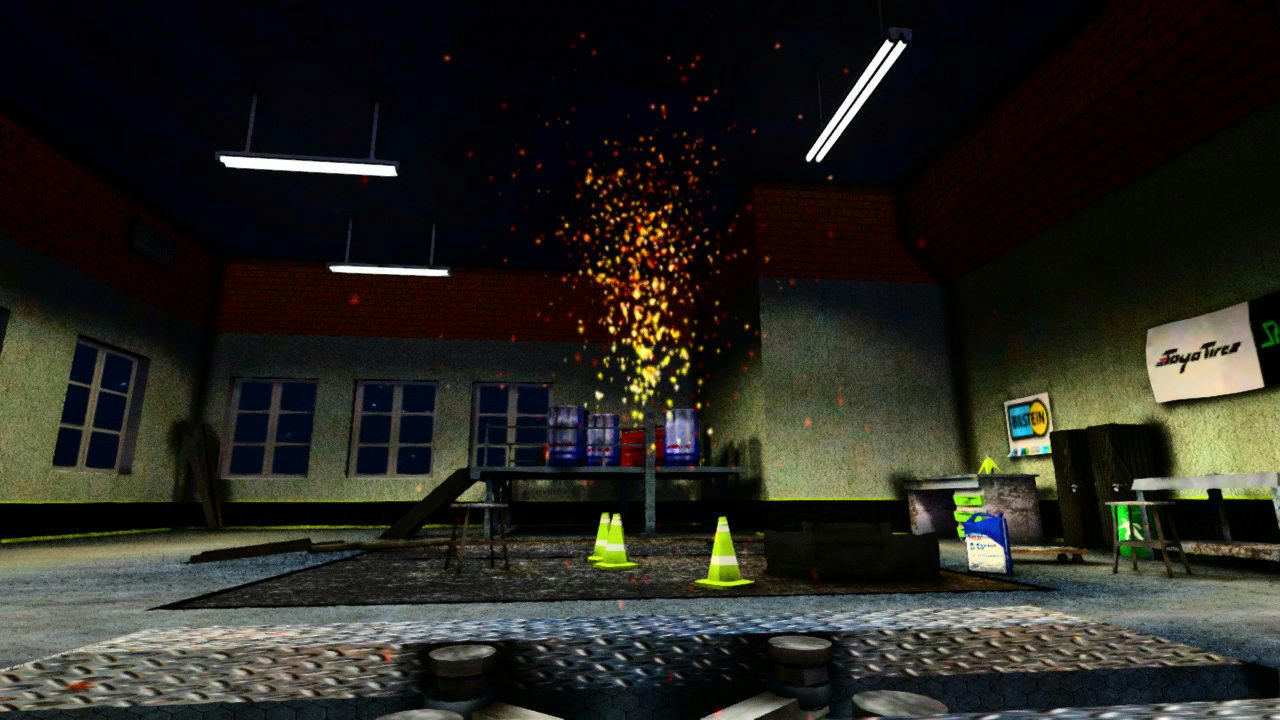```┌─────────────────────────────────────────────────────────────────────────────────────────────────────────────────────────╲
│  RenderRef particleRender = null;
│  ParticleSystem particleRef;
│  Vector particleSystems = new Vector();
│  int particelCounter = 0;
│  {
│  	if(particleRender == null)
│  	{
│  		particleRender = new RenderRef(particles:0x00000106r);
│  	}
│  	particelCounter++;
│  	particleRef = new ParticleSystem(map,particleRender,"scriptParticle"+particelCounter);
│  	particleRef.setSource("particle"+particelCounter, p, 0.01, 0.3, new Vector3(0,4.0,0), 0.0, 1.5, 100000.0, null);
│  	particleRef.setFreq(0.1);
│  }
│  public void enter( GameState prev_state )
│  {
│  	GfxEngine.flush();
│  	super.enter( prev_state );
│
│  }
│  public void exit( GameState next_state )
│  {
│  	for(int i = 0; i != particleSystems.size();++i)
│  	{
│  		particleSystems.elementAt(i).destroy();
│  	}
│  	super.exit( next_state );
│  }
└─────────────────────────────────────────────────────────────────────────────────────────────────────────────────────────╱```
This would have the desired effect (regardless of the particle density setting (of course if it is 0 than there wont be any))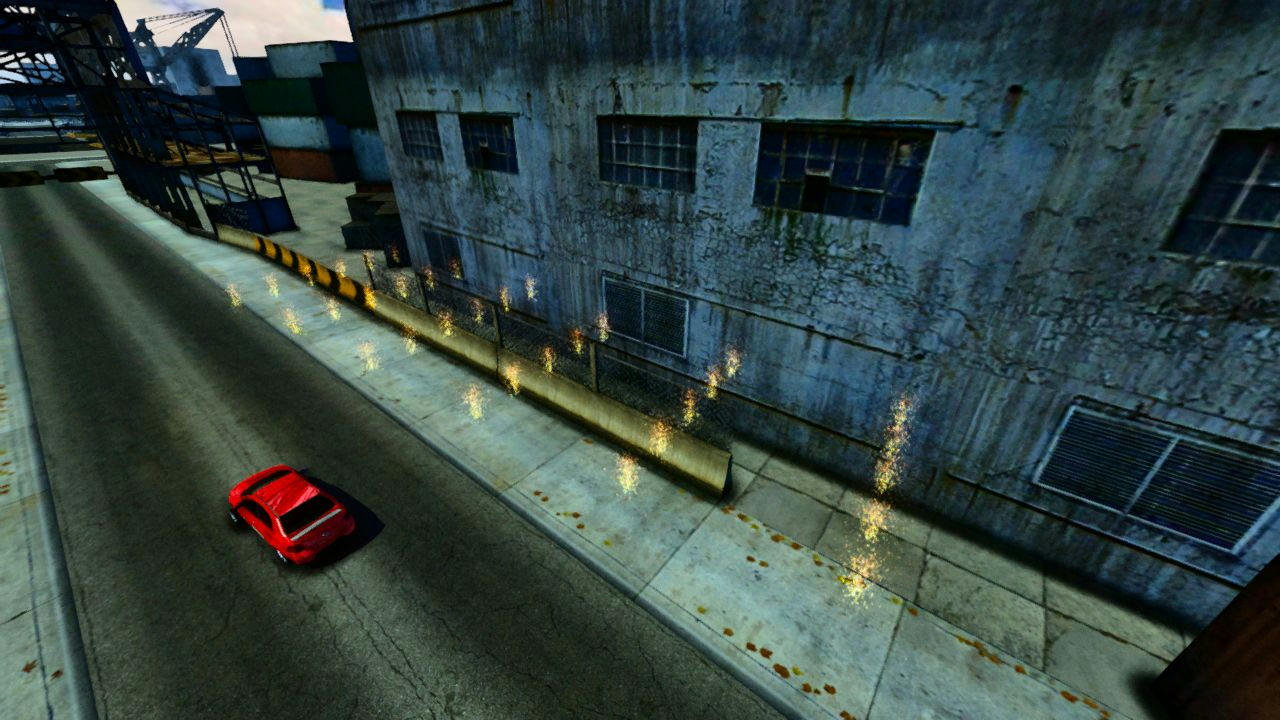You need to save the name of the particle you want to update this functionality is complex enough to warrant a separate class the most basic one can be this.
With this you can call the CreateOrUpdateParticle(..) function every time in the animate block and if you call it with a name already defined it wont create a new particle but instead move it but if the name does not belong to any particle yet it will create a new one.
```┌─────────────────────────────────────────────────────────────────────────────────────────────────────────────────────────╲
│  public class ParticleWrapper
│  {
│  	Vector particleSystems = new Vector();
│  	Vector particleNames = new Vector();
│  	RenderRef particleRender = null;
│  	ParticleSystem particleRef = null;
│  	public void AddParticle(String name,ResourceRef map, int ParticleTypeID
│  				, Vector3 p, float rmin, float rmax, Vector3 vel,
│  				float vmin, float vmax, float freq, float globalFreq)
│  	{
│  		particleRender = new RenderRef(ParticleTypeID);
│  		particleRef = new ParticleSystem(map,particleRender,"scriptParticle");
│  		particleRef.setSource(name, p, rmin, rmax, vel, vmin, vmax, freq, null);
│  		particleRef.setFreq(globalFreq);
│  	}
│  	public void UpdateParticle(String name, Vector3 p, float rmin, float rmax,
│  				Vector3 vel, float vmin, float vmax, float freq, float globalFreq)
│  	{
│  		for(int i = 0; i != particleNames.size(); i++)
│  		{
│  			if(particleNames.elementAt(i) == name)
│  			{
│  				particleSystems.elementAt(i).setSource(name, p, rmin, rmax, vel, vmin, vmax, freq, null);
│  				particleSystems.elementAt(i).setFreq(globalFreq);
│  			}
│  		}
│  	}
│  	public void CreateOrUpdateParticle(String name,ResourceRef map, int ParticleTypeID
│  					, Vector3 p, float rmin, float rmax, Vector3 vel,
│  					float vmin, float vmax, float freq, float globalFreq)
│  	{
│  		for(int i = 0; i != particleNames.size(); i++)
│  		{
│  			if(particleNames.elementAt(i) == name)
│  			{
│  				UpdateParticle(name, p, rmin, rmax, vel, vmin, vmax, freq, globalFreq);
│  				return;
│  			}
│  		}
│  		AddParticle(name,map, ParticleTypeID, p, rmin, rmax, vel, vmin, vmax, freq, globalFreq);
│  	}
│  	public void CleanParticles()
│  	{
│  		for(int i = 0; i != particleSystems.size();++i)
│  		{
│  			particleSystems.elementAt(i).destroy();
│  		}
│  		particleSystems = new Vector();
│  		particleNames = new Vector();
│  	}
│  }
└─────────────────────────────────────────────────────────────────────────────────────────────────────────────────────────╱```
Amilmand
Associate
Posts: 57
Joined: 04 Oct 2019 00:50

SetVel Command

There is a setvel command in Slrr with a format of "%*s %f,%f,%f,%f,%f,%f" the first three floats are the new velocity of the object (preferably a car(vehicle)) the last three are the new ypr and sadly not angular velocity. example:
```┌─────────────────────────────────────────────────────────────────────────────────────────────────────────────────────────╲
│  player.car.command( "setvel 0, 10, 0, 0, 0, 0" );
└─────────────────────────────────────────────────────────────────────────────────────────────────────────────────────────╱```
Amilmand
Associate
Posts: 57
Joined: 04 Oct 2019 00:50

SfxTable

I also added a function to the SfxTable (sparking from adnan54's idea) class which can be used to retrieve the base address of the added sound and with that one can modify the properties of the sound without calling addItem repeatedly, in a more performant manner.
```┌─────────────────────────────────────────────────────────────────────────────────────────────────────────────────────────╲
│  public native int AddItemPtr( ResourceRef sfx, float pitch, float pmin, float pmax, float vmin, float vmax );
│  //these are surely valid offsets (from soundBaseOffset=AddItemPtr(..)):
│  //RawEdit.setF(soundBaseOffset+0x10,1.0/*new_pitch*);
│  //RawEdit.setF(soundBaseOffset+0x14,*unkown*);
│  //RawEdit.setF(soundBaseOffset+0x18,*unkown*);
│  //RawEdit.setF(soundBaseOffset+0x1C,*unkown*);
│  //RawEdit.setF(soundBaseOffset+0x20,*unkown*);
└─────────────────────────────────────────────────────────────────────────────────────────────────────────────────────────╱```
Amilmand
Associate
Posts: 57
Joined: 04 Oct 2019 00:50

I added a new function to the chassis.class
```┌─────────────────────────────────────────────────────────────────────────────────────────────────────────────────────────╲
│  public native int getIndexedAddr( int index );
└─────────────────────────────────────────────────────────────────────────────────────────────────────────────────────────╱```
which acts similar to the getIndexedData but instead of returning the data as float pointed by the offset it returns the offset this coupled with the address of the current gear as an int which is the gear ratio + 0x20 or
```┌─────────────────────────────────────────────────────────────────────────────────────────────────────────────────────────╲
│  public static final int IndexedData_GearNumber = 0x20FC-0x304;
└─────────────────────────────────────────────────────────────────────────────────────────────────────────────────────────╱```
is enough to set the gear to a specific hmm gear the numbering goes as expected but the R is 7.

With an example call of
```┌─────────────────────────────────────────────────────────────────────────────────────────────────────────────────────────╲
└─────────────────────────────────────────────────────────────────────────────────────────────────────────────────────────╱```
the result is:

(mind you I have the ThrottleOnInAutoShiftDeadTime at 1.0 that is why the original shifting results in some throttling this also exemplifies that ofcourse there is some difference in setting the gear like this and through the "controller" (the ingame class not the peripheral) and this can be a limitation there will be no dead time just an instant shift)
If the car is set to automatic in the settings the game will overwrite the changes made to this offset.
Amilmand
Associate
Posts: 57
Joined: 04 Oct 2019 00:50

There is a box trigger "natively" in the game check the source of the Trigger class there is a constructor for it:
```┌─────────────────────────────────────────────────────────────────────────────────────────────────────────────────────────╲
│  public Trigger( GameRef parent, GameRef type, Vector3 pos, float x, float y, float z, String alias)
│  {
│  	if( type == null )
│  		type = new GameRef( DEFAULT );
│          trigger = new GameRef(parent, type.id(), pos.toString() +",0,0,0,box,"+ x +","+ y +","+ z, alias);
│  }
└─────────────────────────────────────────────────────────────────────────────────────────────────────────────────────────╱```
I'm fairly certain that the 3 zeros following the position are the ypr rotation coordinates.
```┌─────────────────────────────────────────────────────────────────────────────────────────────────────────────────────────╲
│  trigger = new GameRef(parent, type.id(), pos.toString() +",y,p,r,box,"+ x +","+ y +","+ z, alias);
└─────────────────────────────────────────────────────────────────────────────────────────────────────────────────────────╱```
So someone can make box triggers with 9 degrees of freedom if needs be.
Here is a function for ease of use (this can be used with the addTrigger function of the track class with the signature:
```┌─────────────────────────────────────────────────────────────────────────────────────────────────────────────────────────╲
│  public Trigger addTrigger( Trigger t, Vector3 pos, RenderRef marker, String handler )
└─────────────────────────────────────────────────────────────────────────────────────────────────────────────────────────╱```
```┌─────────────────────────────────────────────────────────────────────────────────────────────────────────────────────────╲
│  public Trigger MakeBoxTrigger( GameRef parent, GameRef type,
│    Vector3 pos, float x, float y, float z,
│    float yaw, float pitch,float roll, String alias)
│  {
│    Trigger ret = new Trigger(parent,type,pos,alias);
│    ret.trigger.destroy();
│    ret.trigger=null;
│    if( type == null )
│      type = new GameRef( system:0x00000034r );
│    ret.trigger = new GameRef(parent, type.id(), pos.toString() +","+yaw+","+pitch+","+roll+",box,"+ x +","+ y +","+ z, alias);
│    return ret;
│  }
└─────────────────────────────────────────────────────────────────────────────────────────────────────────────────────────╱```
Amilmand
Associate
Posts: 57
Joined: 04 Oct 2019 00:50

Speed limit for the cruise mode

I found a way to set the speed limit for the cruise mode luckily it is set in the AI part of a vehicle which is relatively easy to access here are some lines that can be used to engage the cruise mode and set the speed limit for a given car:
```┌─────────────────────────────────────────────────────────────────────────────────────────────────────────────────────────╲
│  public static final int IndexedData_Flags = 0x70; //these should go in the chassis.java
│  public static final int IndexedData_SpeedLimit = 0x2104;
│  ...
│  int flags = RawEdit.getI(player.car.chassis.getIndexedAddr(Chassis.IndexedData_Flags));//these should go where you want to set the limit
│  flags |= 0x1000;//this sets the cruise mode on to set it to off this line should be flags &= 0xFFFFEFFF
└─────────────────────────────────────────────────────────────────────────────────────────────────────────────────────────╱```
this 1.0 will be the speed limit mind you this is not in kph or anything related its in some arbitrary unit, 13.33 is the fall back value so this 13 should be some theoretical maximum you would need to play with the values to find an optimum. (1.0 is around 5-10 kph)
I mean this will work on tracks that do not define their trc file I did not test it in Valocity but I would presume the original values in the trc file will overwrite direct manipulation with the RawEdit class.
I tried it out the AI cars will stick to the limit set in this way when they are following a spline, and for the player's car (I only tested the player's) the limit will not get overwritten in valocity.
Amilmand
Associate
Posts: 57
Joined: 04 Oct 2019 00:50

Circular nav
Is it possible to turn the small map/gps navigator into a circle instead of a rectangle?
No(the game defines the navigator as a viewport which is rectangular and then tiles the map texture on a very big plane so no not directly)
You can approximate the circle with some (3 in this case) rectangles though of course the error of the approximation induces a wider than "current trends would deem pretty" border.

I created 3 navigators ensuring the correct ratios and relative positions (0.7071 is(~) sin(45°) 0.4871 is(~) -sin(22.5°) 0.8733 is(~) -cos(22.5°)) in the track like:
```┌─────────────────────────────────────────────────────────────────────────────────────────────────────────────────────────╲
│  nav =  new Navigator( -23.482, -24.45, 5.828, maps.city.smallmap:0x00000001r,
│  	maps.city.smallmap:0x00000002r, maps.city.smallmap:0x00000005r, 8, 8, 8 );
│  nav2 = new Navigator( -23.482, -24.45, 5.828, maps.city.smallmap:0x00000001r,
│  	maps.city.smallmap:0x00000002r, maps.city.smallmap:0x00000005r, 8, 8, 8  );
│  nav3 = new Navigator( -23.482, -24.45, 5.828, maps.city.smallmap:0x00000001r,
│  	maps.city.smallmap:0x00000002r, maps.city.smallmap:0x00000005r, 8, 8, 8  );
│  float aspectX = 0.09;
│  float aspectY = 0.16;
│  float scale = 1.78;
│  float initialX = 0.44;
│  float initialY = 2.66;
│  nav.changeSize(  aspectX*scale*(initialX), aspectY*scale*(initialY),
│  	aspectX*scale*(0.7071), aspectY*scale*(0.7071) );
│  nav2.changeSize( aspectX*scale*(initialX+(0.7071-0.4871)/2.0), aspectY*scale*(initialY-(0.8733-0.7071)/2.0),
│  	aspectX*scale*(0.4871), aspectY*scale*(0.8733) );
│  nav3.changeSize( aspectX*scale*(initialX-(0.8733-0.7071)/2.0), aspectY*scale*(initialY+(0.7071-0.4871)/2.0),
│  	aspectX*scale*(0.8733), aspectY*scale*(0.4871) );
└─────────────────────────────────────────────────────────────────────────────────────────────────────────────────────────╱```
The zoom is viewport-height dependent so the animate block also needed updating:
```┌─────────────────────────────────────────────────────────────────────────────────────────────────────────────────────────╲
│  nav.updateNavigator( player.car );
│  nav2.updateNavigator( player.car );
│  nav3.updateNavigator( player.car );
│  nav.cam.setMatrix( new Vector3(CamPos.x/100, GPS_Min_Zoom + Speed, CamPos.z/100),  new Ypr(Rot, -1.57, 0.0) );
│  nav2.cam.setMatrix( new Vector3(CamPos.x/100, (GPS_Min_Zoom + Speed)*1.236, CamPos.z/100),  new Ypr(Rot, -1.57, 0.0) );
│  nav3.cam.setMatrix( new Vector3(CamPos.x/100, (GPS_Min_Zoom + Speed)*0.69, CamPos.z/100),  new Ypr(Rot, -1.57, 0.0) );
└─────────────────────────────────────────────────────────────────────────────────────────────────────────────────────────╱```
Thats basically it.
(I also replaced the GPS frame with a circle with the correct size for 16:9)
Amilmand
Associate
Posts: 57
Joined: 04 Oct 2019 00:50

This was surprisingly easy to do there is a variable in the WheelRef at a small enough offset that actually scales well (if you set it to negative it will invert the steering) this offset is wheelref.ptr+0xc8 and it is a float, I used the RawEdit.setF function to write to it.
(I'm on my laptop so the video is quite choppy but it serves its purpose as a demo)
Amilmand
Associate
Posts: 57
Joined: 04 Oct 2019 00:50

Scalable parts

Sort of, the key is to make a duplicate of the mesh as to not scale all the instances of the same mesh. If you would just do:
```┌─────────────────────────────────────────────────────────────────────────────────────────────────────────────────────────╲
│  int meshID = getMesh();
│  temp_meshRes = new ResourceRef(meshID);
│  temp_meshRes.scaleMesh(2.0,2.0,2.0);
└─────────────────────────────────────────────────────────────────────────────────────────────────────────────────────────╱```
Then if you had the same part two times in an inventory (or anywhere in the game) both would become twice as big.
So the correct would be:
```┌─────────────────────────────────────────────────────────────────────────────────────────────────────────────────────────╲
│  int meshID = getMesh();
│  temp_meshRes = new ResourceRef(meshID);
│  temp_meshRes.duplicate(temp_meshRes);
│  setMesh(temp_meshRes.id());
│  temp_meshRes.scaleMesh(2.0,2.0,2.0);
└─────────────────────────────────────────────────────────────────────────────────────────────────────────────────────────╱```
Another quirk is that this is additive so this:
```┌─────────────────────────────────────────────────────────────────────────────────────────────────────────────────────────╲
│  temp_meshRes.scaleMesh(2.0,2.0,2.0);
│  temp_meshRes.scaleMesh(2.0,2.0,2.0);
└─────────────────────────────────────────────────────────────────────────────────────────────────────────────────────────╱```
will make the mesh 4 times bigger.

DynamicRim with "tune-able" mesh, size and other params (the wheel params that can passed to the SetupTyre(..) function) the result is interesting :) , I also maintained the paintability of the rims with a trick (I made a RenderRef with a texture array consisting of 10 of the same texture which is handily the paintable one, this ofcourse makes all textures the rim uses paintable even the reflection but reshade overwrites that so no harm done (well at least when reshade is active))
(For this(the dynamic mesh selection) to work I had to make a long list of valid mesh TypeIDs and renderRef TypeIDs for tyres and rims so the solution is not hmm portable but it can be done (and if we'll find a way to get the MeshID of a given renderRef in script it can be made portable aswell, nevertheless the method can easily be used for a single modded rim)

I know it looks silly but at least now we CAN make it look dumb without much hassle.

Amilmand
Associate
Posts: 57
Joined: 04 Oct 2019 00:50

First version of HLSL replacement

I came around and replaced the fixed function pipeline implementation of the reflecting surfaces to a programmable one, this is good because it opens up the game for advanced lighting models, though I could not manage to get per-object data to the d3d9.dll from the javascript engine; the rendering and the virtual machine is very well separated, this is bad however because eventhough we now can manipulate the appearance of parts to quite a great extent this feature can not be controlled from the script engine and that makes the whole thing again global (each reflecting surface will have the same properties (at least the same overwrites)).
Another bad thing about this is that because Slrr uses directx in an extremely uninformed manner (a frame in Valocity can easily exceed 60000 d3d calls...) FPS will suffer a bit (it's quite playable in my opinion, and the additional visual fidelity is well worth the 2-7 FPS deficit) and there isn't really anything to be done about it :/ (or at least not with my knowledge)
But now there is a reshadeFx\NativeHLSL\uPS_VS.hlsl file which can be used(and modified) to render the ingame reflecting surfaces (if someone is good with this kind of stuff, I do know that the hunt for the perfect car-paint shader is still interesting to some)

The good part is properly aligned relfections (from 2:30 in the video https://youtu.be/jfszn5efFks?t=164), a nicer specular reflection with "flakes", proper spot lights, per-pixel lighting, bump-mapping I must emphasise that it is bump-mapping not normal mapping (there is no available tangent or bitangent from the model format and the game is preforming slow enough as it is(without generating these at runtime) further more I'm pretty sure my bump map implementation is incorrect but I think there is another matrix needing inverting for it to be pristine but that is time consuming so I opted out)
mainly the ability to modify this aspect of the game is the plus side :)

Before: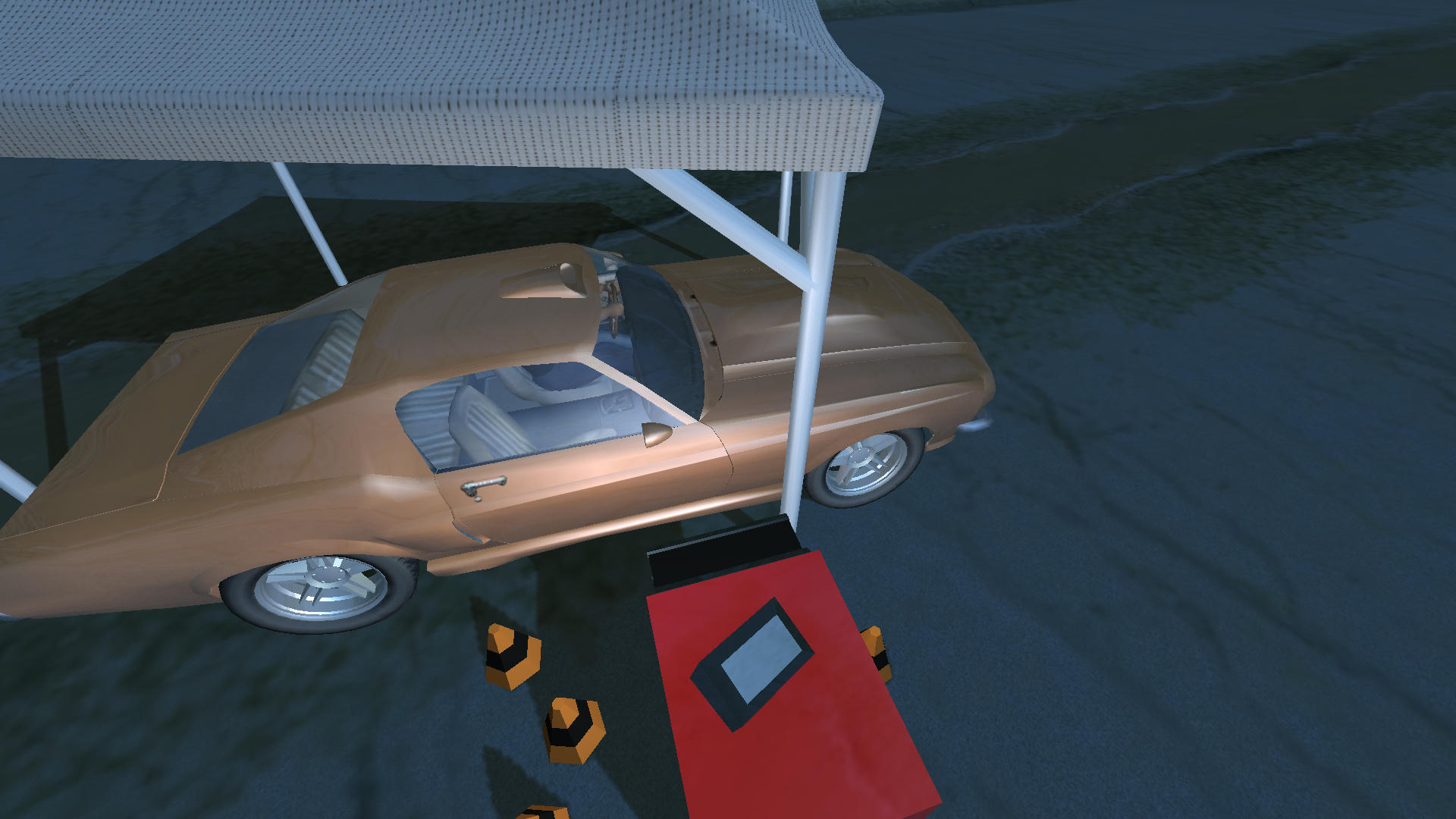After: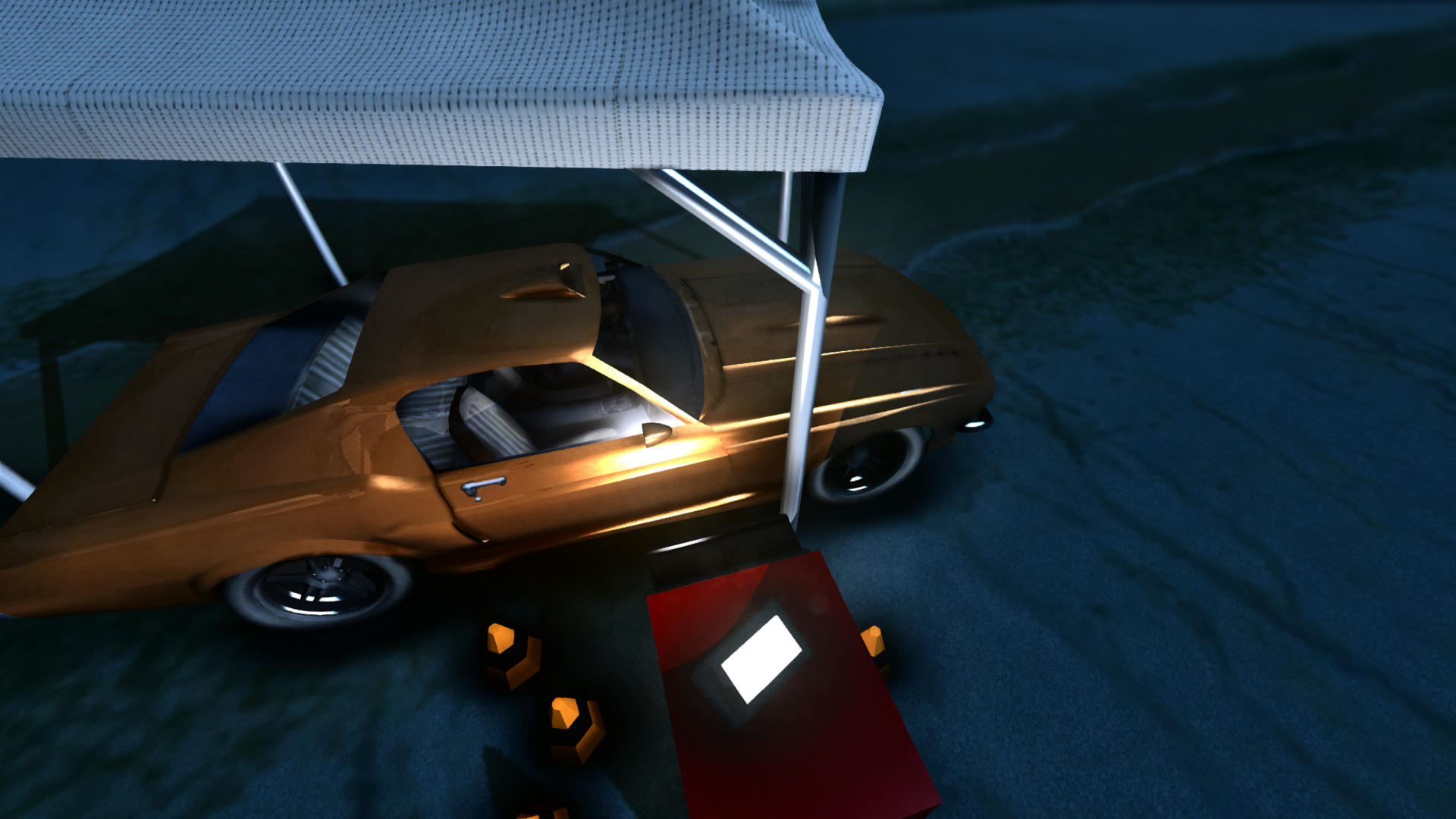Before: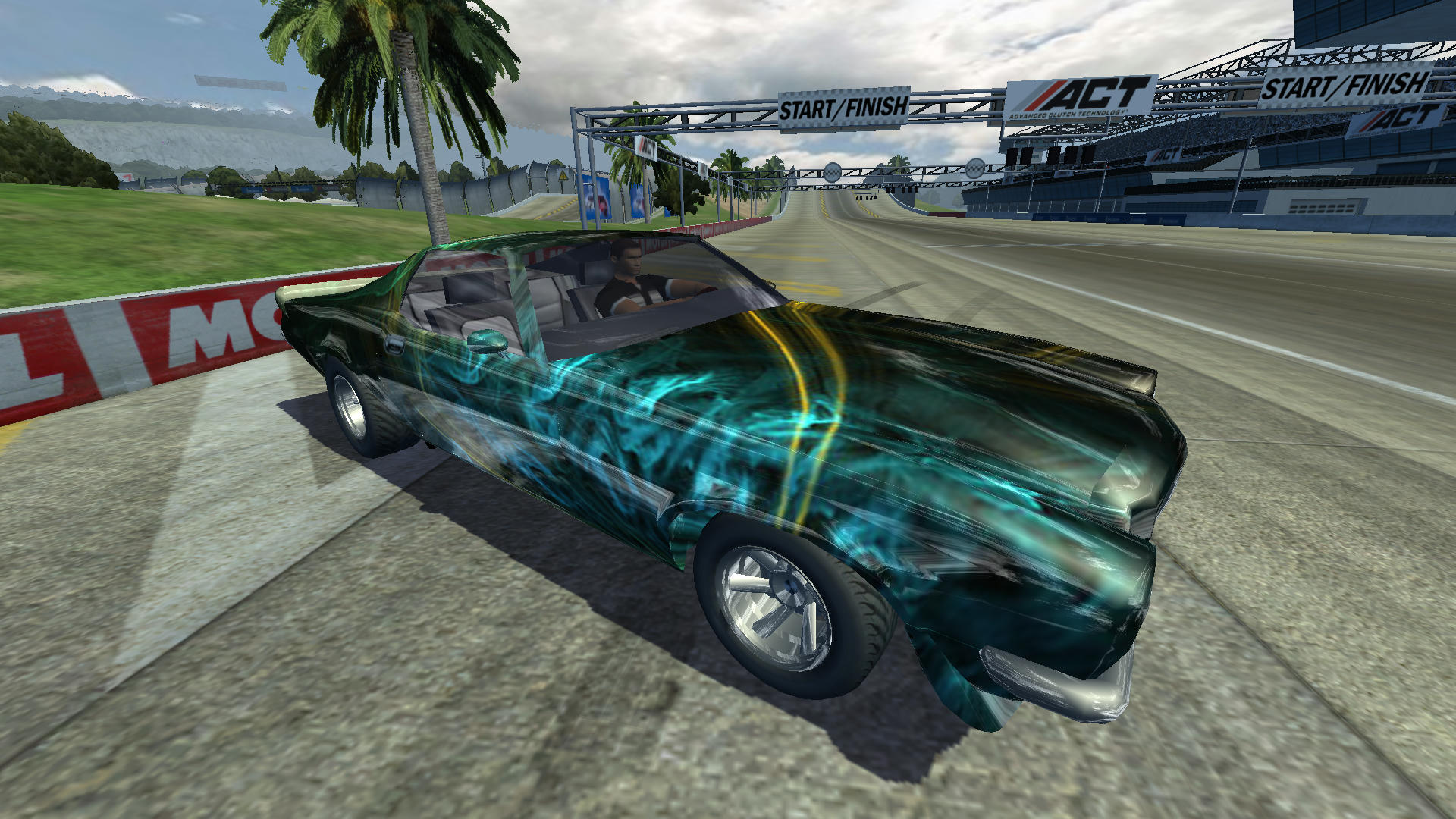After: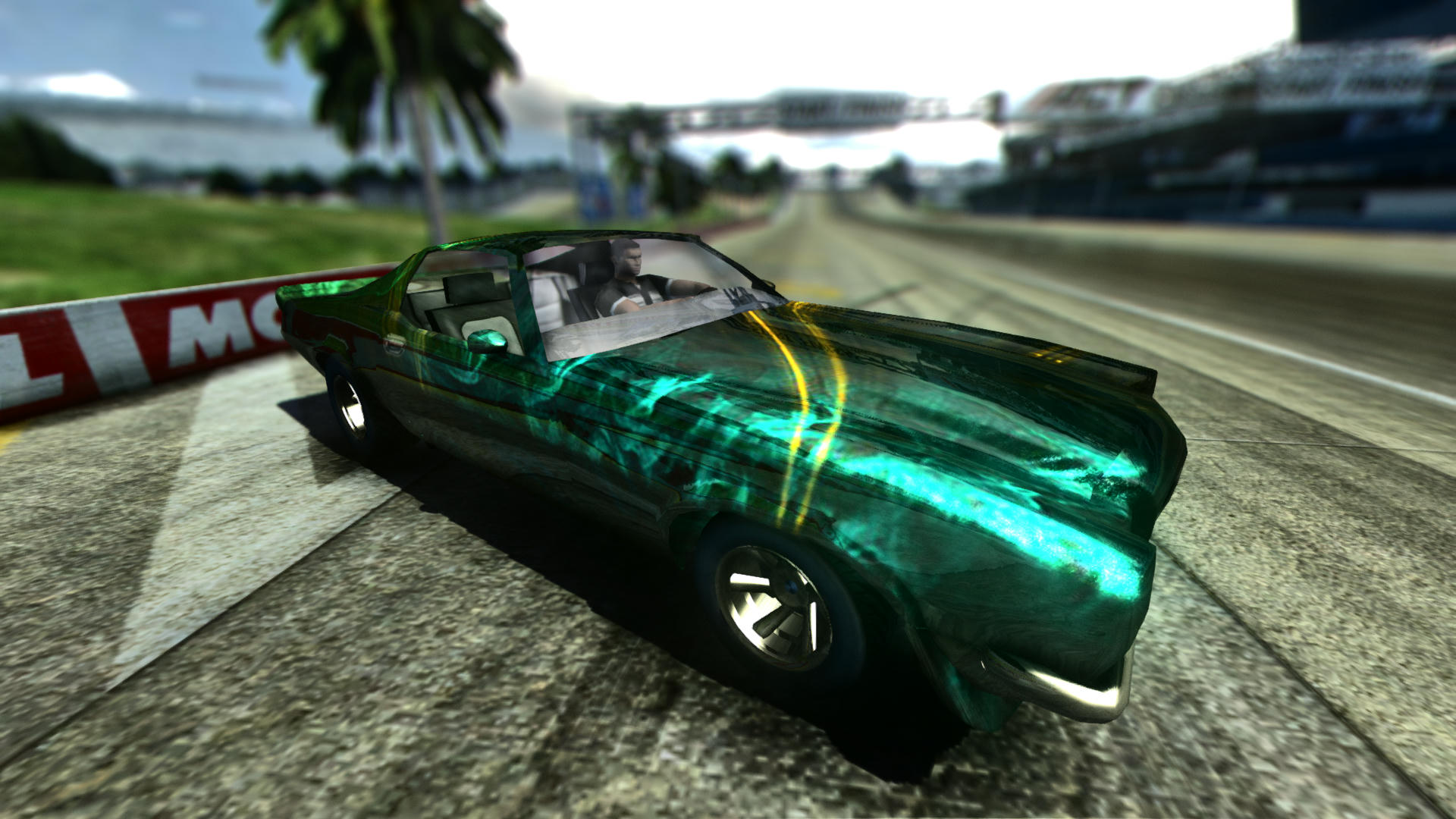Before: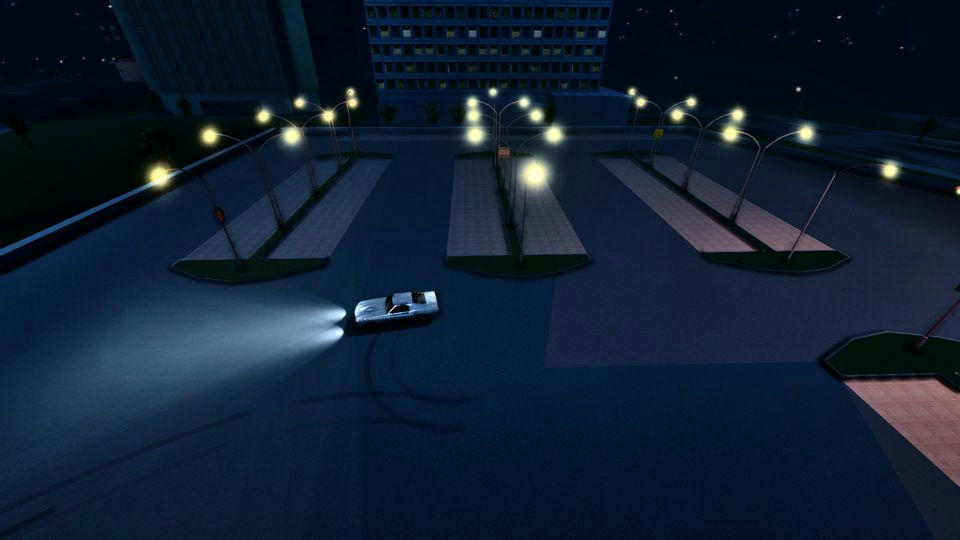After: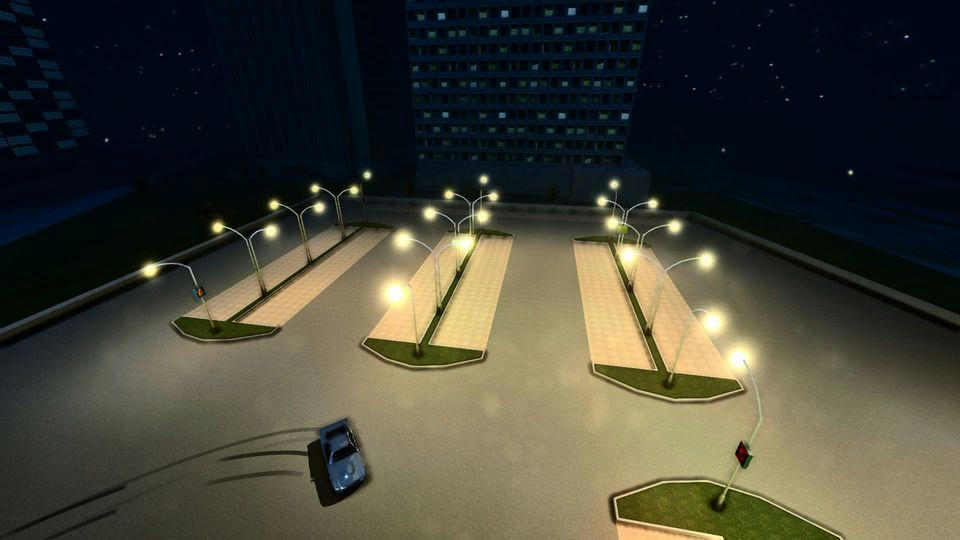Before: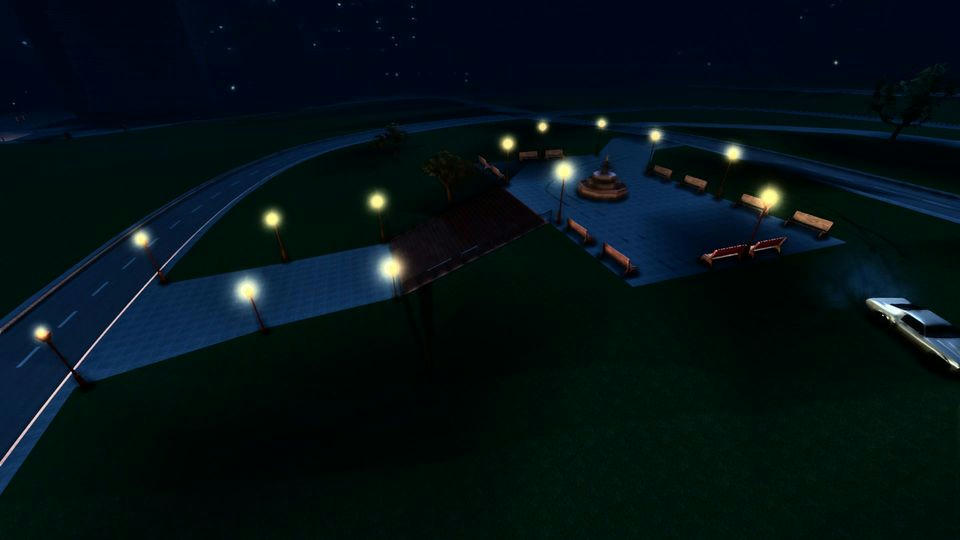After: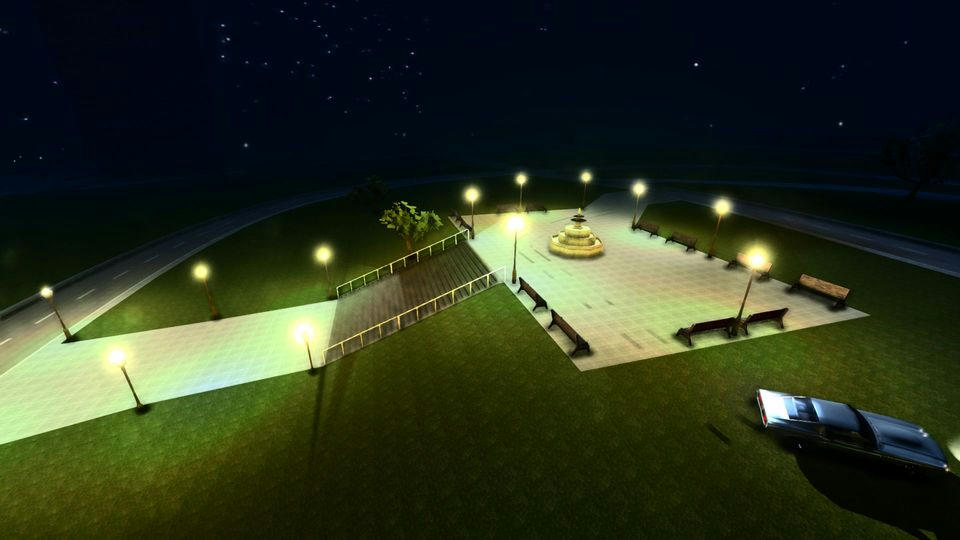LOD (settings from max to min):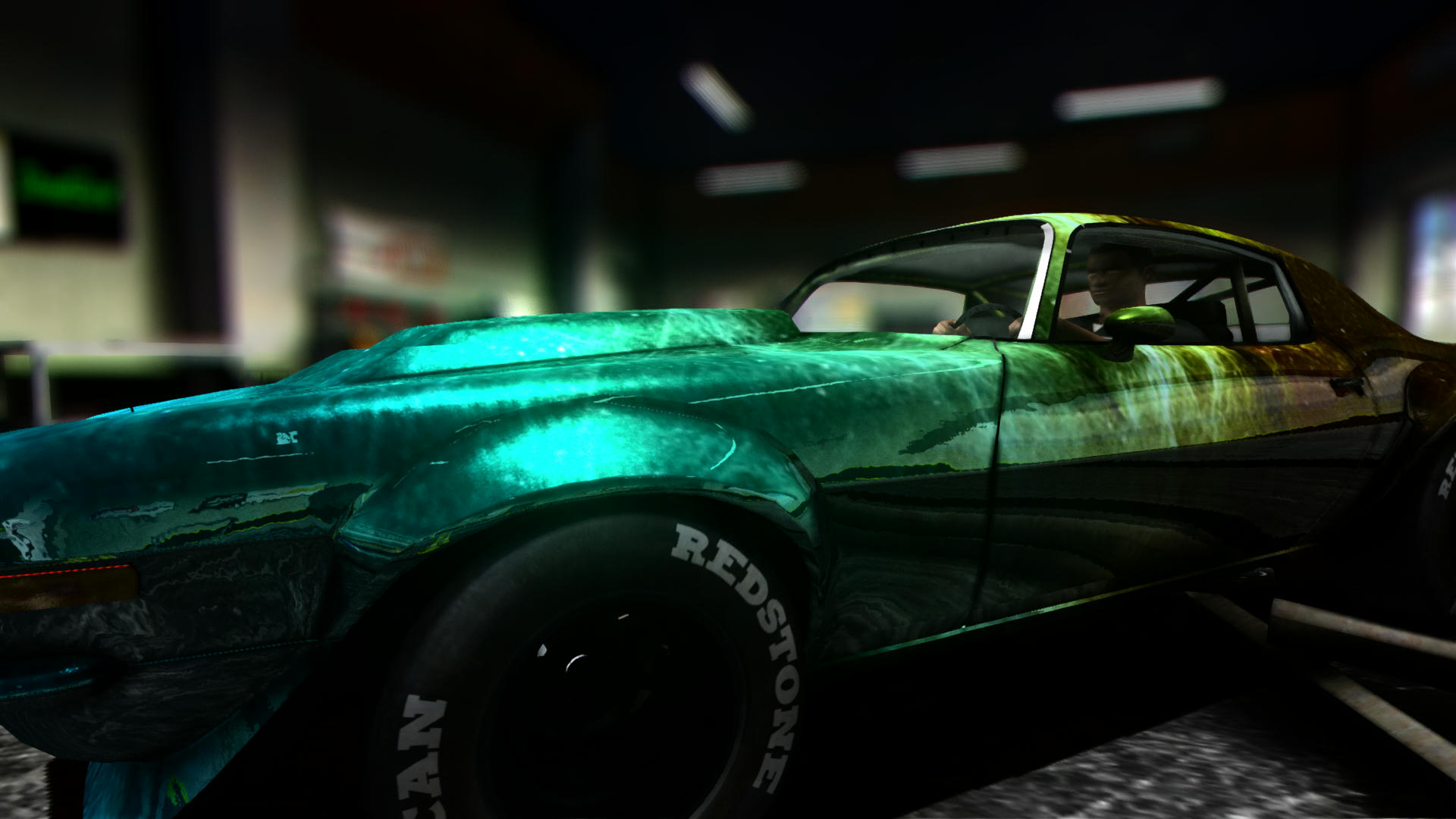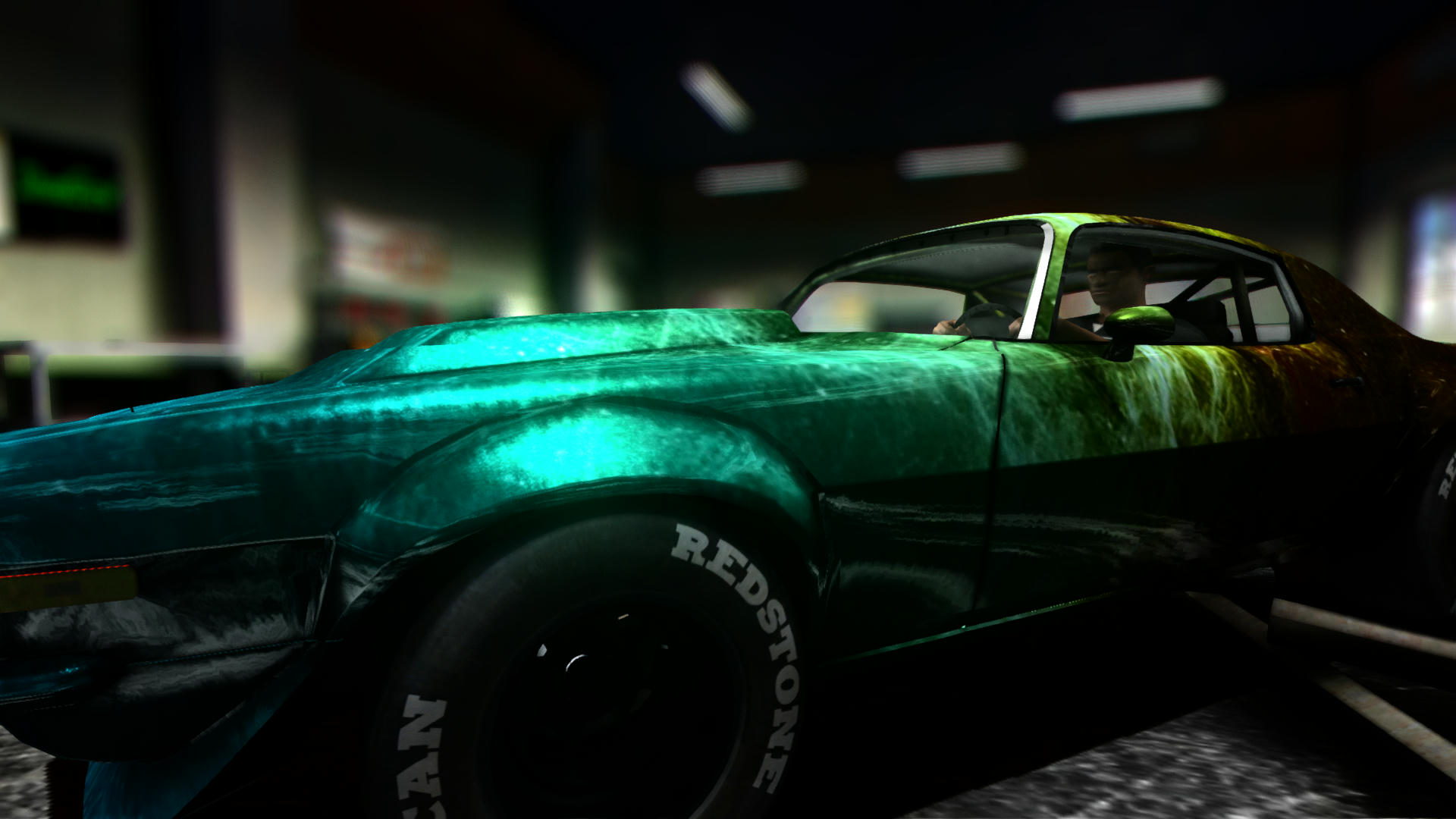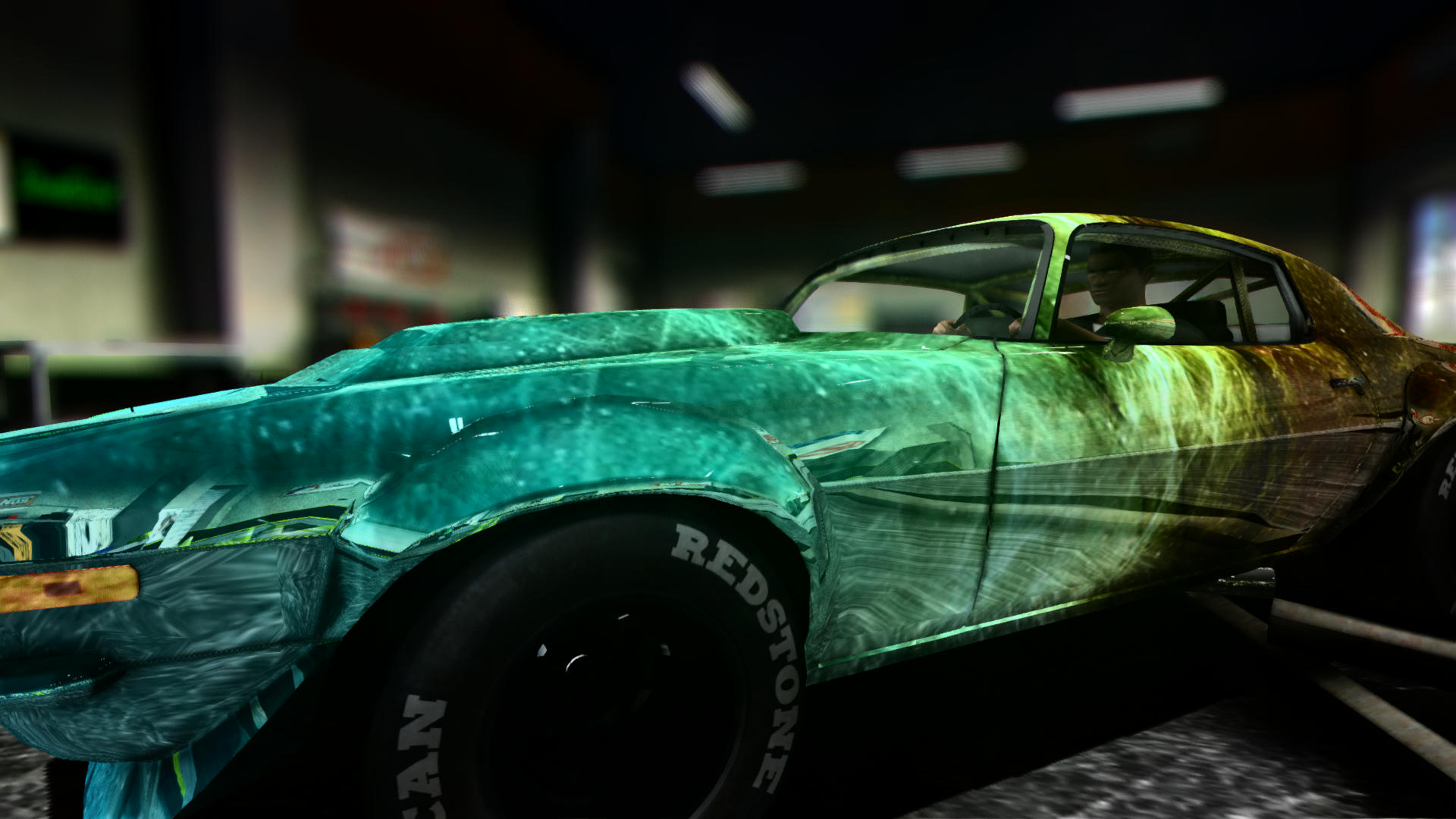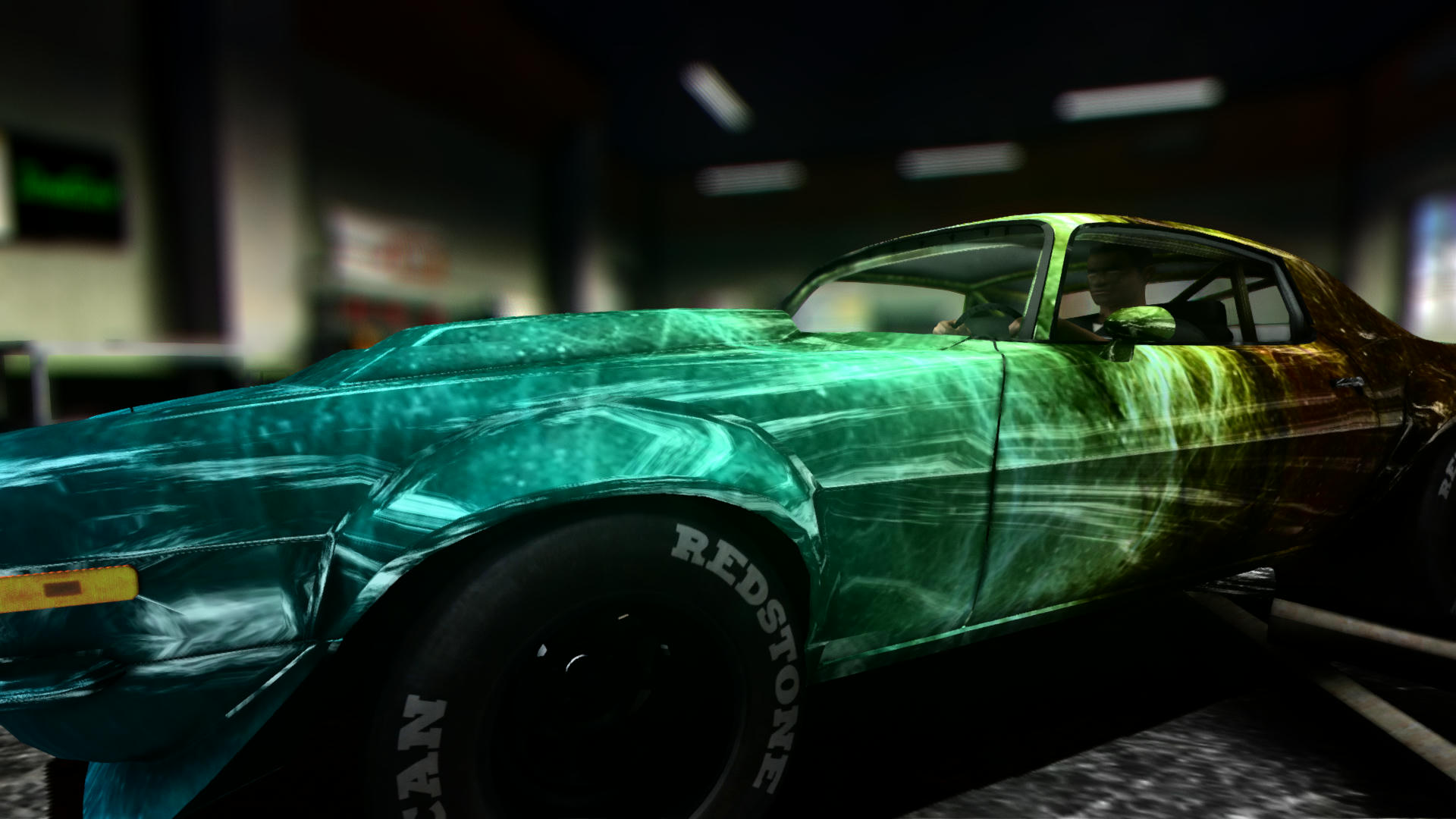(I must admit that my solution feels quite suboptimal but I honestly can't see a meaningful way to improve it this is not my expertise though and I'm fairly certain that if someone who actually knows shader programming takes a look at the .hlsl will faint let's hope after waking up he/she will optimize the code a bit and share the results :D )
Of course the current implementation is highly customisable as it has been before, there are numerous new properties in the ReflectionSettings.fx in the Reshade menu (for once the name checks out)

Another thing about the reflecting surface shader replacement has a hack; if the texture drawn as the diffuse layer 1 has a pure #FF00FF top left corner (for the shader to get a pure #FF00FF there must be a large pink border around the image because texture filtering blurs it) it treats it as a rusted texture zooms it a bit as to not render the pink border part and lessens the reflection on the surface making it more like a rusted part and not a nice rustlike paintjob with polish and everything.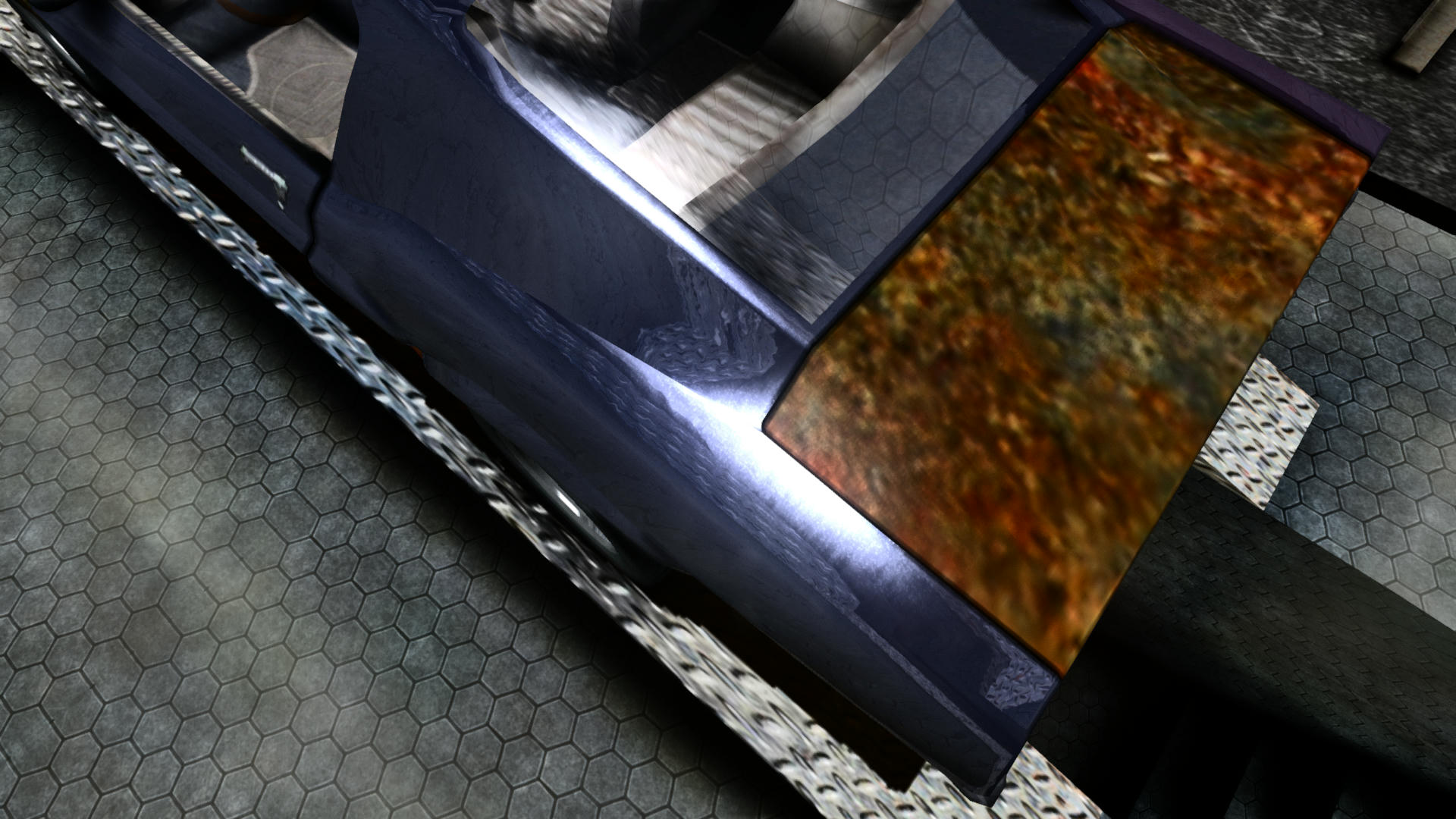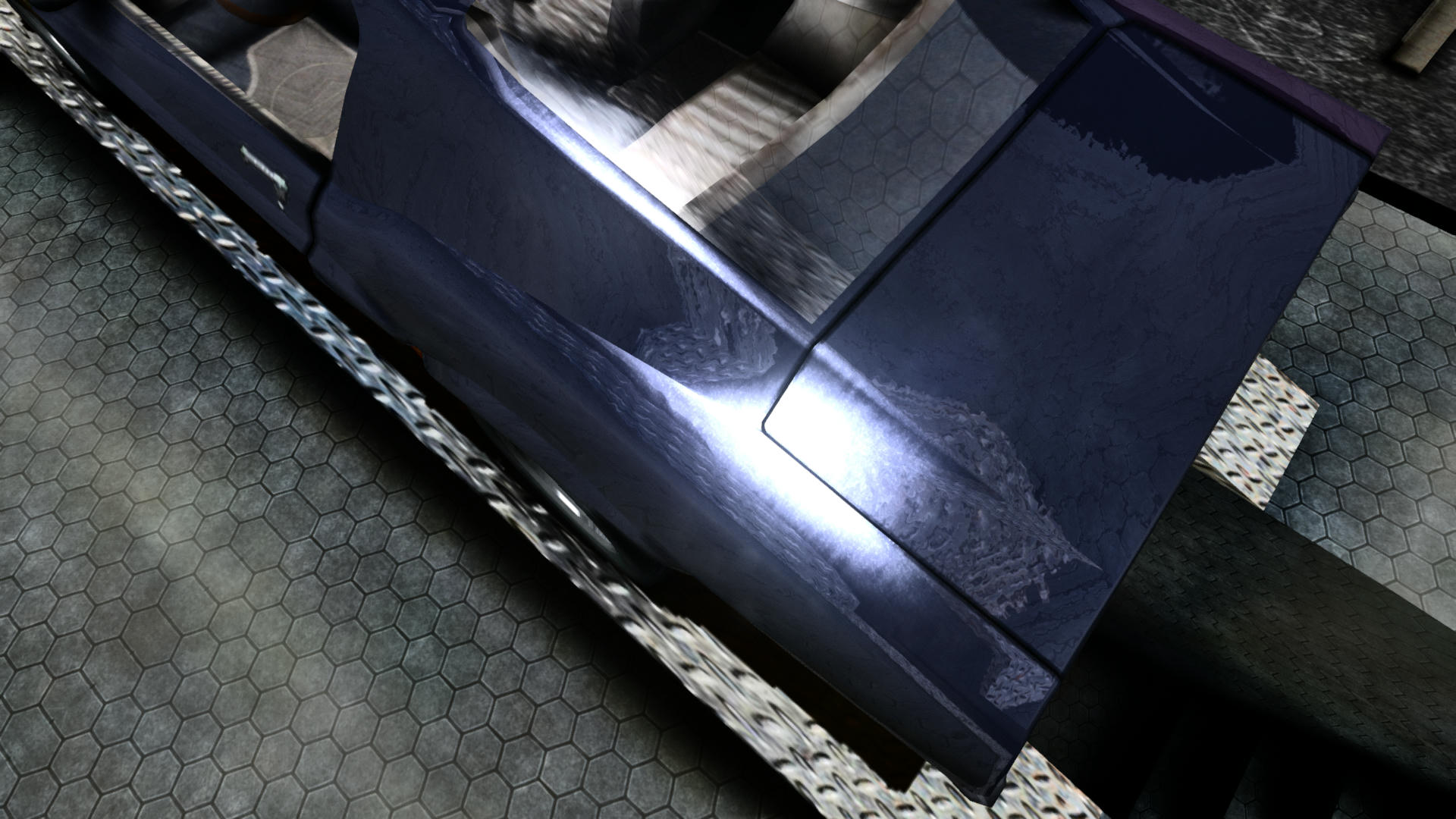Been thinking about it and you can potentially experience longer loading times because the game does not unload objects in regard to frustum culling anymore and the initial position of the camera does affect the time the loading screen is up (if you have obscenely high draw distance this can be more noticeable)
but this is for good reason and it has great benefit regarding fps: here is a comparison video (we can see that the original (left) unloads objects(the car, buildings) and spends a lot of time reloading them when they eventually get into the view frustum which results in atrocious fps:

I added a Fresnel factor for the reflection to tone it down a bit.

Before: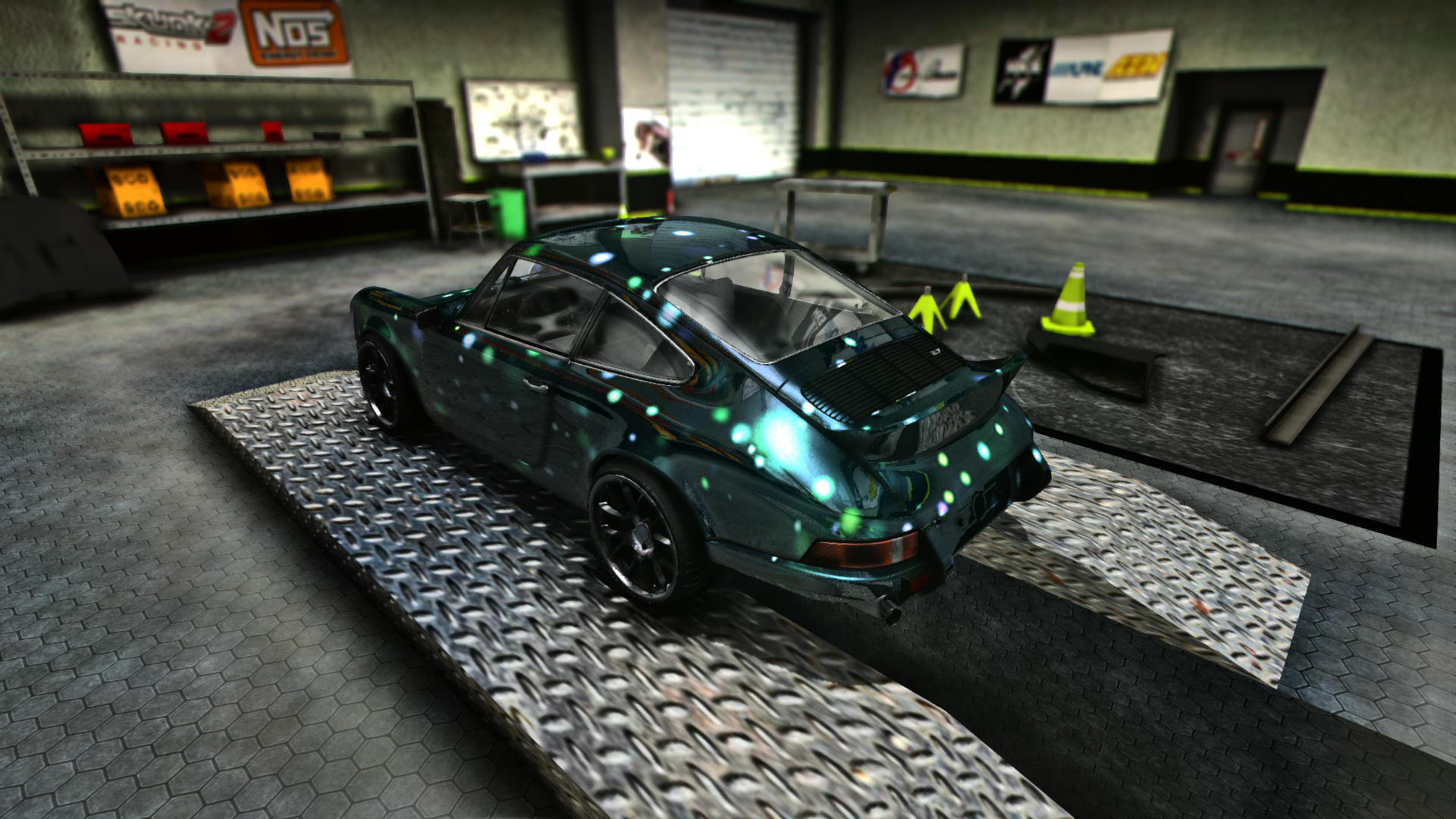After: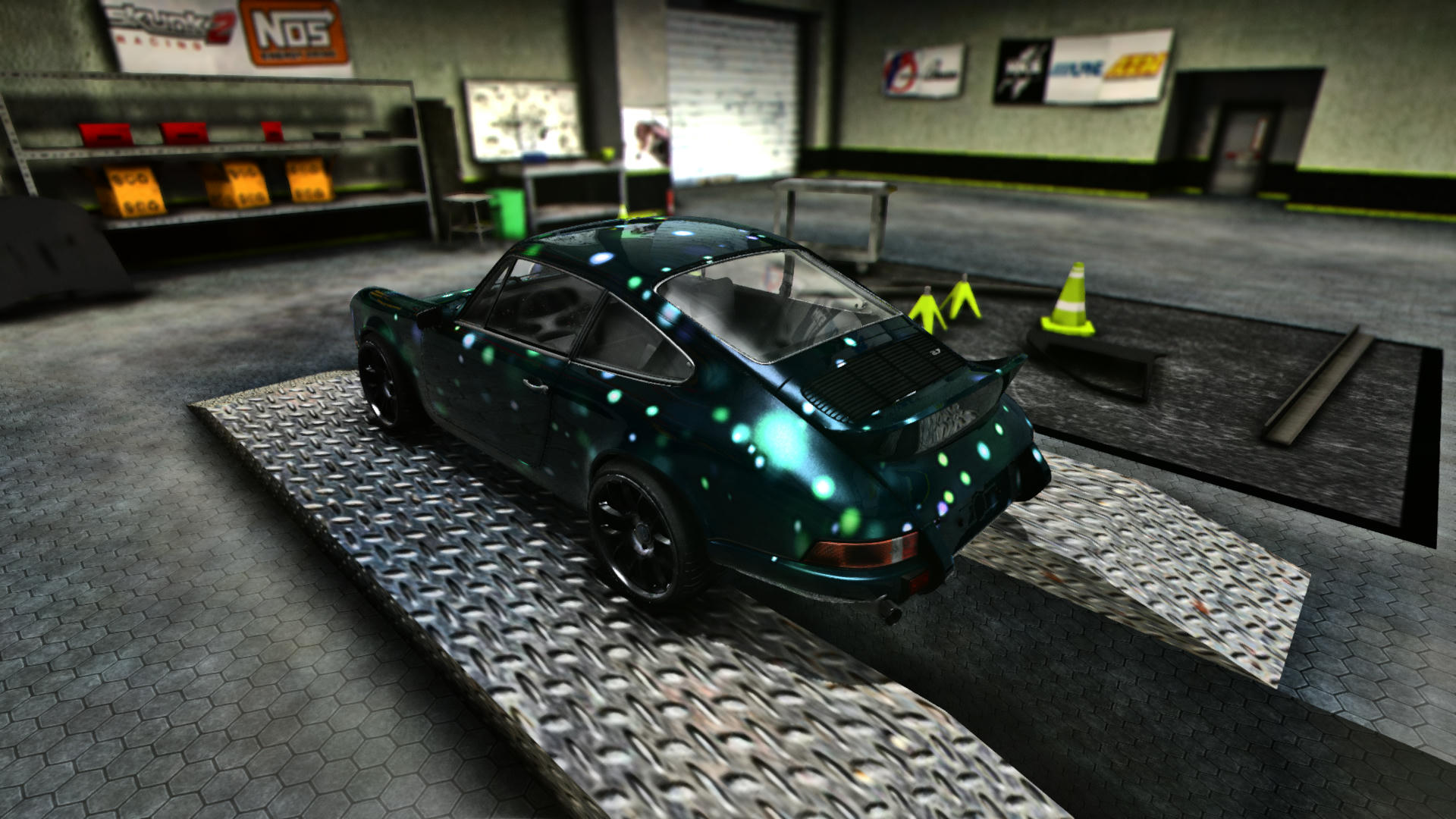Amilmand
Associate
Posts: 57
Joined: 04 Oct 2019 00:50

Multi RPK tracks manually

I've been thinking about this and there are tools out there (namely this: viewtopic.php?f=52&t=13247) that you can use to edit map-rpks directly long story short you can make a two(or more) rpk map with this from two normal map-rpks you create in the usual way (track wizard or something like that):

You should decide on a parent map-rpk (this will be the one you put the player into with a new GroundRef( ... ); call in a Track)
The child rpk(s) should be edited:
-Turn the map into an rdb2 with the tool above
-Add the parent rpk to the external references
-Set the SuperID of the GroundRef definition in the child rpk to
the GroudnRef definition in the parent rpk (these (GroundRefs) are usually the 0x00000001 TypeIDs in modded maps)
-Set the IsParentCompatible to 1
-Turn the edited rsd2(s) into an rpk and replace the original

That is basically it not a big hassle (the position of the maps should be set in 3dsmax to line up nicely they will both be placed with the same origin)
(You can check out the alignment with any of the MapEditor programs I posted by choosing Render and opening the parent rpk and adding the others with AddRender)

Here is a quick example adding LimeRockPark to the WeekendDrive map:

Amilmand
Associate
Posts: 57
Joined: 04 Oct 2019 00:50

Car state flags
Hey Amilmand, sorry to bother you, but I had a question: do you know how to get the car's current flags? I've tried both getFlags(); and (int)the_car.getIndexedData(Chassis.IndexedData_Flags); and neither worked.
Almost there but as you found out (judging by the static cast to int) this function returns a float soo its no good for you the full call would be to get the address of the value and decode it as an int:
```┌─────────────────────────────────────────────────────────────────────────────────────────────────────────────────────────╲
└─────────────────────────────────────────────────────────────────────────────────────────────────────────────────────────╱```
The comments in the chassis.java could have helped:
```┌─────────────────────────────────────────────────────────────────────────────────────────────────────────────────────────╲
│  public static final int IndexedData_GearNumber = 0x20FC-0x304;
│     //this is an int getIndexedData will return
│       strange but the RawEdit.getI with getIndexedAddr will suffice
│  public static final int IndexedData_Flags = 0x70;//int
└─────────────────────────────────────────────────────────────────────────────────────────────────────────────────────────╱```
I dont know what your goal is with the flags but I found that access to this value is of a readonly manner as most of the flags the game updates each frame and invalidates any changes you try to force (though you can get interesting state indicators so to say, (whether the blinker is on, is the car running and some others)

As for checking function availability, you cant read arbitrary data from files so the actual function name is problematic but you can check for files present, ExhaustiveBits depends on the RawEdit class so you could check for
```┌─────────────────────────────────────────────────────────────────────────────────────────────────────────────────────────╲
│  File.exsits("system\\Scripts\\lang\\RawEdit.class");
└─────────────────────────────────────────────────────────────────────────────────────────────────────────────────────────╱```
as a reasonable indicator, still if the game is not run with the Slrr_GI.exe you are hooped :/ I haven't made a way to detect that.
But there is no general check in the default game you can make to decide whether a function name is valid or not (or I dont know about it).
I was afraid it was going to be impossible to read the car's flags well enough to tell whether or not the headlights (or indicators, etc) are on
Well this is not entirely true you can check for whether the car thinks its night time (this is the same flag that gets checked by the lights) and check for the rpm if its >0 if so the lights are most probably on, there is also a flag for the indicators (but of course for that to actually get flipped the cruse mode needs to be on) and all this is read only as the game overwrites changes.
Code would look along the lines:
```┌─────────────────────────────────────────────────────────────────────────────────────────────────────────────────────────╲
│  float rpm = GameLogic.player.car.chassis.getIndexedData(Chassis.IndexedData_RPM);
│  if((flags & 0x8) != 0 && rpm > 1)
│  {
│    //lights are most probably on
│  }
│  if((flags & 0x10000) != 0)
│  {
│    //left indicator blinking (mind you this is true for the whole time its in the blinking state not just when its actually lit)
│  }
│  if((flags & 0x20000) != 0)
│  {
│    /right indicator
│  }
└─────────────────────────────────────────────────────────────────────────────────────────────────────────────────────────╱```
But really you dont even need this, you can check for the steering with
getIndexedData(Chassis.IndexedData_CurrentSteering1) (the game does the same for the built in method)
for the indicators, (even enforce them when cruise mode is inactive).
And the current game time also can be acquired (the game checks for (currentTime < 75600 && currentTime >= 21600)) along with the rpm.
(and again the whole shabang for brake lights, IndexedData_BreakStrength, or clucth lights (if that is a thing) IndexedData_ClutchStrength ooor nitro lights IndexedData_NitroStrength and alot others)

If I'm already writing I'll mention that I found a way to detect whether the car is flying or not (to be more precise I found a value in the WheelRef instance that is usually close to 0.0 when the car is in the air (it reports 0 if the game disables collision on the car (by the suspend command for example) it can also happen when the car is not moving so there is a constraint on it but its not that bad) :
```┌─────────────────────────────────────────────────────────────────────────────────────────────────────────────────────────╲
│  WheelRef whl;
│  float sumPressure = 0.0;
│  for(int whl_i = 0; whl_i != 4; whl_i++)
│  {
│  	whl = player.car.chassis.getWheel(whl_i);
│  }
│  if(sumPressure <= 0.001)
│  {
│  	//player car is probably flying...
│  	Vector3 carVel = player.car.getVel();
│  	carVel.y = 0;
│  	if(carVel.length() > 5)
│  	{
│  		//player car is most probably flying...
│  	}
│  }
└─────────────────────────────────────────────────────────────────────────────────────────────────────────────────────────╱```

Each wheel's speed individually?
```┌─────────────────────────────────────────────────────────────────────────────────────────────────────────────────────────╲
│  heelRef whl = player.car.chassis.getWheel(0);//the order used by the stock cars: 0:front_left 1:front_right 2:rear_left 3:rear_right (I think)
│  RawEdit.getF(whl.ptr+0xC0);
└─────────────────────────────────────────────────────────────────────────────────────────────────────────────────────────╱```
Will tell you a value proportional to the angular velocity of the front left wheel (if the given chassis has that one defined first).
This value is something that you can also set to add torque to the wheel: minuses will make the wheel rotate as to move the car forward and positive values will make the car go in reverse.
The value set manually with the RawEdit.setF function will fall off quickly so you would need to set it continuously for longer distances (to test it a value in the range of 1000 is enough to nudge a car a bit backwards:
```┌─────────────────────────────────────────────────────────────────────────────────────────────────────────────────────────╲
│  WheelRef whl = player.car.chassis.getWheel(0);
│  RawEdit.setF(whl.ptr+0xC0,1000);
└─────────────────────────────────────────────────────────────────────────────────────────────────────────────────────────╱```
This may be of interest to some other people too maybe
Gorgoil asked for a way to set the max rpm more dynamically than to set it on the block and then updatevariables the chassis:

Ok I figured it out I think
The actual max rpm the game uses is in the runtime dyno data there is a pointer to it on the offset 0x1FBC from the base native chassis pointer the 6th float is the max rpm in the runtime dyno data
so to query:
```┌─────────────────────────────────────────────────────────────────────────────────────────────────────────────────────────╲
└─────────────────────────────────────────────────────────────────────────────────────────────────────────────────────────╱```
to set:
```┌─────────────────────────────────────────────────────────────────────────────────────────────────────────────────────────╲
└─────────────────────────────────────────────────────────────────────────────────────────────────────────────────────────╱```
Amilmand
Associate
Posts: 57
Joined: 04 Oct 2019 00:50

The file types of Slrr

I worked a lot to decode the data files (scx,rpk..) and I developed some proof-of-concept
(they do not have, how should I put it, proper production code quality
(if I would have done this at work, well let's just say code review would have been quite a shaming))
little programs using the knowledge, this includes: (available from the main SlrrExhaustive download)
-Spl editor and map viewer (testing the concepts of RPK loading, and the spline .spl files define)
-Extra Part aligner (this includes putting together the car as Slrr would through slot attachments)
-UV editor (also, putting the car together properly so that the projected textures would line up)
-The map editor can load maps in POLY or PHYS editor mode which can be used to move
around vertices in a map or its collision
These programs are not made for general public use but I did not want to withhold
anything they may come in handy for someone someday.
The general model for the datafiles is usable the sourcecode gets problematic in the tools.
This was done in (old)c# because well it was easier, also all the sources are provided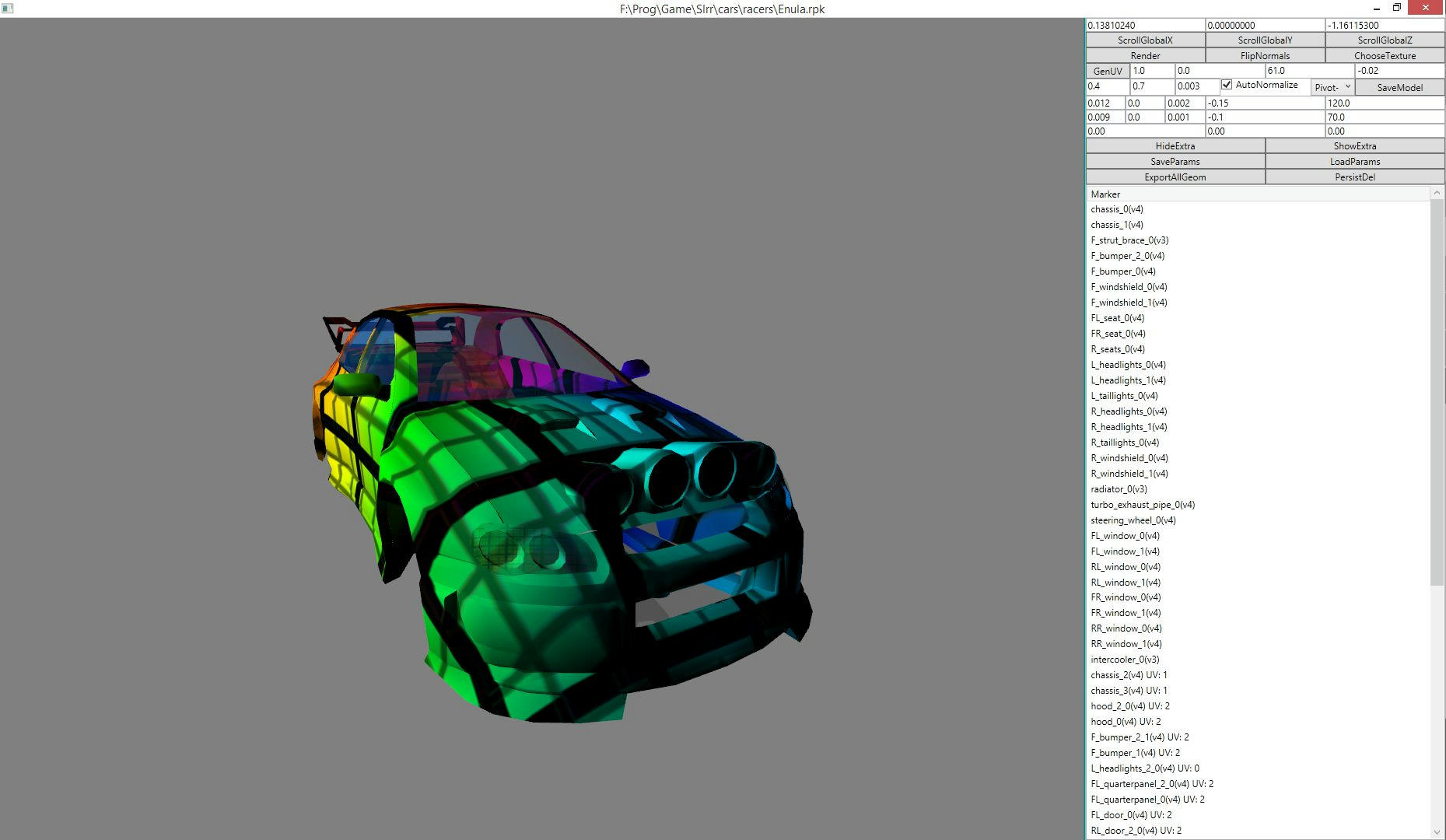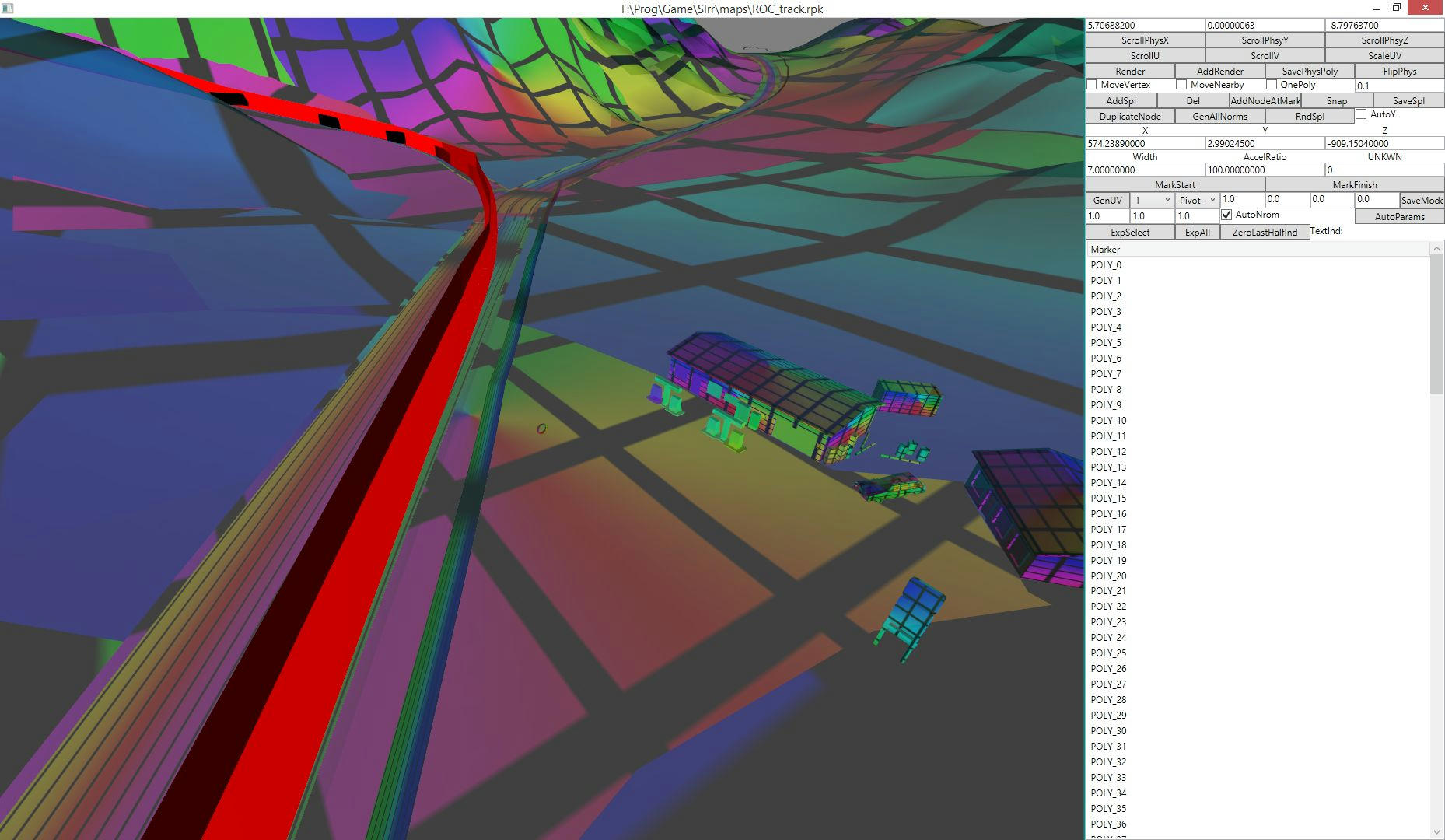On this page I will provide the data file structures (as much as I found out)
which was used to implement the tools
(the c# source for datafile manipulation is understandable and serves as an example
implementation (not all exceptions and common errors are listed here but the c# source is tested)).
source for file manipulation
```Scx files:
There are two versions that Slrr uses for storing 3D model data:
Scx v3 file structure (fields in order of appearance (in a valid file)):
ScxV3
{
char Signature = "INVO"
int Version = 3
ModelDefV3[] models //as many as can be also there may be an int
at the end of the file that is 0
}
ModelDefV3
{
MaterialV3
int vertexCount
VertexDataV3 vertexData[vertexCount]
int faceCount //triangle count
int IndicesV3[faceCount*3]
}
MaterialV3
{
int size

float DiffuseColorR
float DiffuseColorG
float DiffuseColorB
float Opacity

float SpecularColorR
float SpecularColorG
float SpecularColorB
float SpecularColorWeight
float GlossinessWeight

int Flags
{
int flagAlphaOpacity = 0x00000001;
int flagDiffuseBlend = 0x00000100;
int flagVertexColorBlend = 0x00000002;
int flagLayer2VertexColorBlend = 0x00001000;
int flagLayer2BlendByAlpha = 0x00000040;
}

short diffuseMapIndex //Which texture corresponds to which mapindex is set in the
RenderRef definition part as a list of texture typeIDs in a RPK file referencing the given scx file
short bumpMapIndex
short specularMapIndex
short reflectionMapIndex
short diffuseLayer2MapIndex
short unkownMapIndex2
short illuminationIndex
short unkownMapIndex3

int oneVertexSize //in the next VertexDataV3

int unkownInt1
short unkownShort1

short diffuseMixFirstMapChannel  //Channel is the index of UV that should be used
short diffuseMixSecondMapChannel
short bumpMapChannel
short specularMapChanel

short unkownShort2
int unkownInt2
int unkownInt3
int unkownInt4

float illuminationColorR
float illuminationColorG
float illuminationColorB

char materialName
}
VertexDataV3// the size of this part is set in the MaterialV3 and can differ
depending on the fields defined but the order of them is fixed so if an
UV channel 3 should be defined all the field above must be as well
{
float vertexCoordX
float vertexCoordY
float vertexCoordZ
float vertexNormalX
float vertexNormalY
float vertexNormalZ

float uVChannel1X
float uVChannel1Y
float uVChannel2X
float uVChannel2Y

byte vertexColorB
byte vertexColorG
byte vertexColorR
byte vertexColorA

int unkown1
int unkown2

float uVChannel3X
float uVChannel3Y

int unkown3
}
Scx v4 file structure (fields in order of appearance (in a valid file)):
ScxV4
{
char Signature = "INVO"
int Version = 4
implicitly declares the logical connection between entries
(a mesh can only have one material definition...)
byte data[] //header entries point into this
}
{
int entryType
{
FaceIndices = 5,  //FaceDef
MaterialDef = 0,  //MaterialV4
HardSurfaceVertexDefinition = 1,  //HardSurfaceDef
VertexData = 4,  //VertexDataV4
SparseBoneIndexList = 2,  //BoneList
BoneIndexList = 3  //BoneList
}
int entryOffsetInFile
}
MaterialV4
{
int unkownZero = 0
int size //of block
int flags
int unkownZero2 = 0
int EntriesCount
MaterialEntry entries[EntriesCount]
}
MaterialEntry
{
ushort unknownFlag
ushort Type
byte data[DataSize]
//DataSize is dependant on the Type:
//Type == 2048 => DataSize = 32 (char)
//Type == 0 => DataSize = 4 (byte RGBA)
//Type == 256 => DataSize = 4 (float intensity)
//Type == 1536 => DataSize = 28 (MapDefinitionEntry)
//Type == 1280 => DataSize = 4 (int)
//You can also deduce semantic meaning
(for this I used the TypeSwaped = (ushort)((Type >> 8) | (Type << 8)))
//if (unknownFlag == 0 && TypeSwapped == 0)
//  return "Diffuse Color";
//if (unknownFlag == 2 && TypeSwapped == 0)
//  return "Illumination Color";
//if (unknownFlag == 1 && TypeSwapped == 0)
//  return "Specular Color";
//if (unknownFlag == 0 && TypeSwapped == 1)
//  return "Glossiness";
//if (unknownFlag == 1 && TypeSwapped == 1)
//  return "Reflection Percent";
//if (unknownFlag == 2 && TypeSwapped == 1)
//  return "Bump Percent";
//if (unknownFlag == 0 && TypeSwapped == 6)
//  return "Diffuse Map";
//if (unknownFlag == 1 && TypeSwapped == 6)
//  return "Diffuse Mix Second";
//if (unknownFlag == 2 && TypeSwapped == 6)
//  return "Bump Map";
//if (unknownFlag == 3 && TypeSwapped == 6)
//  return "Reflection Map";
//if (unknownFlag == 4 && TypeSwapped == 6)
//  return "Illumination Map";
//if (unknownFlag == 0 && TypeSwapped == 8)
//  return "Material Name";
}
MapDefinitionEntry
{
int textureIndex  //this index is taken from the texture TypeID list defined
in a RPK that references the scx file containing this map definition record
int mapChannel  //UV used
int TillingFlags//3=UTileVTile 0=UMirrorVMirror
float TillingU
float TillingV
float OffsetU
float OffsetV
//if the next entry is a float intensity MaterailEntry it is the
weight of this Map (like reflection strength)
}
FaceDef
{
int type
int size //of block
int numOfShorts
ushort indices[numOfShorts]//I must point out that these are ushorts meaning if there are
more than 0xffff (65535) vertices in a given vertexDataV4 indices can overflow which in
turn can lead to crashes because of the extreme triangles
//well actually slrr even used these as shorts when projecting decals onto parts
(on collision or by painting) so there could only be 0xffff/2 vertices in a mesh
(a model is made out of meshes)
//technically:
//004F2DA1 (offset in Slrr.exe): two loops looping through all indices and hence
all vertices this can calculate bad pointers...
//the loop checks a triangle (3 offsets are calculated but MOVSX is used to
load EDI which is "singextend" meaning it is cast to a short from a ushort....
this is very bad EDI can move backwards(even before the base pointer) in the buffer
if the index is greater than MAX_USHORT/2 which can happen ... that's not that many vertices,
all 6 (3 in each loop) MOVSX instructions should be MOVZX (zero extend))
}
VertexDataV4
{
int type
int size //of block
int numberOfVertices//will be indexed with ushorts in FaceDef so it should not be > 0xffff
int vertexType//flags declaring which fields are defined in the VertexData
//PossibleFeatures: (in order of possible appearance)
//Position = 0x1, float XYZ floats
//BoneWeightNumIs0 = 0x2, 0 weight-float written (one is implicit 1-Sum(WrittenWeights) = 1)
//BoneWeightNumIs1 = 0x4, 1 weight-float written (one is implicit 1-Sum(WrittenWeights))
//BoneWeightNumIs2 = 0x8, 2 weight-floats written (one is implicit 1-Sum(WrittenWeights))
//BoneWeightNumIs3 = 0x10, 3 weight-floats written (one is implicit 1-Sum(WrittenWeights))
//BoneIndRef = 0x20, byte (four indices to the BoneIndexList)
//Normal = 0x40, float XYZ floats
//VertexIllumination = 0x80, byte RGBA
//VertexColor = 0x100, byte RGBA
//UV1 = 0x200, float UV
//UV2 = 0x400, float UV
//UV3 = 0x800, float UV
//BumpMapNormal = 0x40000, float XYZ floats
byte vertexData[(size - 16)] //vertex data defined in accordance with the vertexType
}
HardSurfaceDef //if this entry is present the current mesh is a hard surface mesh meaning
there won"t be any bone references in the vertexDatas
{
int type
int size
int unkownInt = 0
}
BoneList
{
int type
int size
int unkownInt = 0
int BoneIndexList[((size - (3 * 4)) / 4)]
}

Rpk files:
These files are used to define the relations between other data files
(telling a Part script which cfg to use and which scx to use with which textures...)
Rpk
{
byte rsdData[]
}
{
char signature = "RPAK"
int version512 = 512
int externalReferencesCount
int externalReferencesUnkownZero = 0
}
ExternalReference
{
short unkownIndexZero = 0
short indexOfReference
char referenceString //padded with 0-s (at the end)
}
{
int entriesSize;//resEntries size in bytes
int entriesCount
int entriesType1Count // number of entries with typeOfEntry == 1
int entriesNonType1Count
}
ResEntry
{
int superID
int typeID// it is called Type ID because it only needs to be unique in
combination with the value of typeOfEntry
byte typeOfEntry
float isParentCompatible
int fileOffsetOfRSD
int RSDLength
byte aliasLength
char alias[aliasLength] //despite the length defined this must be zeroterminated
(size includes the zero)
(//optional
float floatBounds// it is present if additionalType & 1 != 0
this is used in the spatial data structure used to define maps
)
}
RSDEntry
{
InnerRsdEntry innerEntries[]//as many as the corresponding RSDLength can hold
}
InnerRsdEntry can be one of; ICFGInnerEntry, XCFGInnerEntry, RSDInnerEntry,
StringInnerEntry, EXTPInnerEntry, NamedDataArray, InnerPolyEntry, InnerPhysEntry
ICFGInnerEntry //Internal cfg entry: a cfg file defined directly in the rpk
{
char signature = "ICFG"
int lengthOfData
char cfgData[lengthOfData]// \0 delimited list of strings (2 strings observed)
defining a common cfg with a key
}
XCFGInnerEntry //reference cfg
{
char signature = "XCFG"
int lengthOfData
char cfgData[lengthOfData]// \0 delimited list of strings the first one is usually
empty the second one is a key, a whitespace and than a file path relative to the
slrr root directory to a cfg file
}
RSDInnerEntry
{
char signature = "RSD\0"
int lengthOfData
char stringData[lengthOfData]// string data used mainly to define map "entry points"
//expect NamedDatas and the spatial structure definition of a map (as in track not as in picture)
}
StringInnerEntry
{
char stringData[] //as much as the current RSD entry can hold
(ResEntry.RSDLength (or as much as is left of it))
}
EXTPInnerEntry
{
char signature = "EXTP"
int lengthOfData = 4
int data//only 3 observed it is also the number of the root NamedDataArrays defined
but that can be coincidence
}
NamedDataArray
{
char signature = "\0\0\0\0"
int numOfNamedDatas
NamedData namedDatas[numOfNamedDatas]//these represent the spatial tree data structure
(not a BSP, some axis aligned thing not exactly an octree but definitely some bounding volume hierarchy)
}
NamedData
{
int TypeID
int SuperID
byte typeOfEntry
float isParentCompatible
int offset//in file, references NamedDataArray, InnerPolyEntry or InnerPhysEntry entries
not always just one but uniquely; only one NamedData references a given array or poly or phys
int sizeAtOffset
byte nameLength
char name[nameLength]//just as with the RES entries
float boundingBoxX//in range -50000 .. 50000 positioning the centre of the box
float boundingBoxY//in range -50000 .. 50000
float boundingBoxZ//in range -50000 .. 50000
float boundingBoxHalfWidthX//in range 0 .. 50000 generally small values
float boundingBoxHalfWidthY//in range 0 .. 50000 generally small values
float boundingBoxHalfWidthZ//in range 0 .. 50000 generally small values
float unkownData7// only 0 seen
float unkownData8// only 0 seen
float unkownData9// only 0 seen
float unkownData10// only 0 seen
float unkownData11// only 0 seen
float unkownData12// only 0 seen
}
InnerPolyEntry
{
char signature = "POLY"
int size
int unkownCount1
int meshCount
Mesh meshes[meshCount]
}
Mesh
{
int textureIndex
int verticesCount
int triangleCount
VertexData vertexData[verticesCount]
int Indices[triangleCount*3]
}
VertexData
{
float coordX
float coordY
float coordZ
float normalX
float normalY
float normalZ
byte colorR
byte colorG
byte colorB
byte colorA
byte illuminationR
byte illuminationG
byte illuminationB
byte illuminationA
float uVChannel1U
float uVChannel1V
float uVChannel2U
float uVChannel2V
float uVChannel3U
float uVChannel3V
}
InnerPhysEntry
{
char signature = "PHYS"
int size
int indexChunkCount
IndexChunk indexChunks[indexChunkCount]
int vertexChunkCount
VertexChunk vertexChunks[vertexChunkCount]
}
IndexChunk
{
int unkownInt1//probably the material...
int triIndices
float normalX
float normalY
float normalZ
}
VertexChunk
{
float vertexX
float vertexY
float vertexZ
}

Class files:
The compiled script files of slrr this uses a custom java implementation
it was developed with Flex(,Bison) ... if someone wants a challenge (to investigate the saved AST)
I only figured out the bare minimum that is needed to change the rpk references,
class and package names in the .class files without .java sources
Class
{
TUFA definedClasses[]//sequentially
}
TUFA
{
char signature = "TUFA"
int unkownData1
int unkownData2
SignaturedSizedScriptEntry entries[]//as many as can be till the next TUFA entry,
can be SignaturedSizedScriptEntry or CONSEntry
}
SignaturedSizedScriptEntry
{
char signature
int size;
byte data&#91;size]
}
CONSEntry
{
char signature = "CONS"
int size;
int entriesCount
ConstantEntry entries[entriesCount] //can be ConstantEntry, NameConstantEntry or RpkRefConstant,
the full class name(including the package) is the first NameConstantEntry
the full parent class name is the second
}
ConstantEntry
{
int ID
byte[] data// size is determined by the ID:
//ID == 0 => size = (the first int in data)+4
//ID == 4 => size = 4
//ID == 7 or 3 or 5 => size = 8
//data is generally an int[] referencing other ConstantEntries by their indices in the
given constant table if flatted out it will give a function reference or other string
}
NameConstantEntry
{
int ID = 0
int stringLength
char name[stringLength]
}
RpkRefConstant
{
int ID = 3
int RPKnameIndexInConstantTable//the index of a NameConstantEntry in the current
CONS that will be the rpk referenced (the file path)
int TypeIdInRPK
}
```

In the cfgs for vehicles and parts stockpart 0x00000001 line means that when creating
a dummy model try and attach the part with typeID 0x00000001 (this is used when
creating the cars roaming the city) creating a dummy car:
```┌─────────────────────────────────────────────────────────────────────────────────────────────────────────────────────────╲
│  dummycar = new GameRef();
│  dummycar.create_native( map, new GameRef(dummyID), "0,-10000,0,0,0,0", "dummycar" );
└─────────────────────────────────────────────────────────────────────────────────────────────────────────────────────────╱```
here stockpart references will be attached

In the cfgs for vehicles and parts the texture 0x00000001 line means that the texture 0x00000001
(which should be in the textureID list of the corresponding renderRef definition) will be replaced
when painting is applied (or the setTexture method)
this can be used to create parts with paintable reflection or illumination textures which is quite cool.

Slrr manages RenderRefs competently but scripts are difficult for it this is the reason
why in the demonstration "modded almost to hell" version of Slrr I created all the RenderRef lookup tables
once a script is loaded (meaning an instance of one of the defined classes is created) it can not be unloaded
and this is why slrr will always crash eventually; it is a 32bit application so it can only address
~4Gb memory which will easily run out because every part has a unique class,
of course as demonstrated in the videos this can be pushed out significantly
(using the renderRef lookups), and this is why all the ExtraParts use the same script.

The silent crashes (ones that do not produce a line in the error.log) are
(if you can rule out the obvious missing resource thing and class redefinition
(which can be easily checked using the file type model))
almost always caused by
the GC freeing up a script instance from under another script-thread,
multi threading is very dangerous in Slrr
(Miran's added little camera icon to the navigator
(which is updated from a thread created by the Track class) was a frequent cause of crashes).

The game uses standard fixed function pipeline directx9

The .spl file spline row format (%LF is float %d is int) is:
(%lf %lf %lf) (%lf %lf %lf) %lf %lf %d %lf %lf %d:
(pos XYZ), (normal XYZ), splineWidth, targetVelocity, I do not know the rest
the two ints are used as bools
the list of first two vectors define a closed cubic Hermite spline.

Slrr can not load more than 256 RPKs and there are at least 5 reserved slots that get filled by the exe
itself and not by loading a library this modded version loads 248 (I think), and that is pretty much the maximum
possible.
Jesus Christ
The Godfathers
Posts: 398
Joined: 11 Apr 2017 12:19
Contact:
Nice to see some progress in this! Still ripping it apart haha!Wish some new modders jumped in to replace us retired ones.Why be a KING when you can be a GOD?!
Amilmand
Associate
Posts: 57
Joined: 04 Oct 2019 00:50
Yeah I hear you :) that would be nice
Amilmand
Associate
Posts: 57
Joined: 04 Oct 2019 00:50
I have found a way to directly edit the weights of a car on the cfg level meaning that if you edit the weights of a Prime then all the Primes will have the same weights, I also devised a way to do this on the car level meaning that it become a normal configurable attribute of a chassis like the wheel positions but that is for Exhaustive only (the order of the body lines matter and I rely on custom ASM code in the Exhaustive version of the Slrr_GI.exe):

To edit the n-th body line in the runtime cfg structure (which is a reversed level order traversal of the tree structure of the car):
```┌─────────────────────────────────────────────────────────────────────────────────────────────────────────────────────────╲
│  int GetNthPhysPointer(int target_ind)
│  {
│  	int phys_base_ptr = RawEdit.getI(base_chassis_ptr + 0x13BC);
│  	int phys_inner_ptr = RawEdit.getI(phys_base_ptr + 0x24);
│  	float weight = RawEdit.getF(RawEdit.getI(phys_inner_ptr + 0x5C)+0x14);// this is the reciprocal of the weight written in the cfg
│  	int ind = 0;
│  	while(phys_inner_ptr != 0)
│  	{
│  		phys_inner_ptr = RawEdit.getI(phys_inner_ptr + 0x28);
│  		if(phys_inner_ptr != 0)
│  			weight = RawEdit.getF(RawEdit.getI(phys_inner_ptr + 0x5C)+0x14);// this is the reciprocal of the weight written in the cfg
│  		if(ind == target_ind)
│  			return phys_inner_ptr;
│  		ind++;
│  	}
│  	return -1;
│  }
└─────────────────────────────────────────────────────────────────────────────────────────────────────────────────────────╱```
This value gets persisted whenever the car is given away to the physics engine this means that if the car is moving or woken up this way of editing the weights wont work but I also found the dynamic weight that the physics engine uses.
To set the dynamic weight to 7000Kg:
```┌─────────────────────────────────────────────────────────────────────────────────────────────────────────────────────────╲
│  int dynamic_phys_ptr = RawEdit.getI(base_chassis_ptr)-0x18;
│  int inner_phys_ptr = RawEdit.getI(dynamic_phys_ptr)+0x5C;
│  int dynamic_weight_ptr = RawEdit.getI(inner_phys_ptr)+0x14;
│  RawEdit.setF(dynamic_weight_ptr,1.0/7000.0);
└─────────────────────────────────────────────────────────────────────────────────────────────────────────────────────────╱```
Associate
Posts: 18
Joined: 01 Oct 2019 19:34
holy f*ck thats so awesomeSomething wrong is not right.
Amilmand
Associate
Posts: 57
Joined: 04 Oct 2019 00:50
I found a way to find substrings in other strings in Slrr which was surprisingly missing until now, its quite simple though as the ptr filed of a string variable is the actual zero terminated char array corresponding to that string.
After that you only need a function to get a single byte from an arbitrary address which we can construct from the RawEdit.getI class and some bit manipulation.
So the substring function looks like this:
```┌─────────────────────────────────────────────────────────────────────────────────────────────────────────────────────────╲
│  public int ContainsSubstring(String search_in,String search_for)
│  {
│  	int ind_in = 0;
│  	int ind_for = 0;
│  	while(val_in != 0 && val_for != 0)
│  	{
│  		while(val_in == val_for)
│  		{
│  			ind_in++;
│  			ind_for++;
│  			if(val_for == 0)
│  			{
│  				return 1;
│  			}
│  		}
│  		if(ind_for != 0)
│  		{
│  			ind_for = 0;
│  		}
│  		else
│  		{
│  			ind_in++;
│  		}
│  	}
│  	return 0;
│  }
└─────────────────────────────────────────────────────────────────────────────────────────────────────────────────────────╱```
Where the byte from int function is a simple bitwise and with 0x000000FF like so:
```┌─────────────────────────────────────────────────────────────────────────────────────────────────────────────────────────╲
│  {
│  }
└─────────────────────────────────────────────────────────────────────────────────────────────────────────────────────────╱```
To make a proper filter or search functionality, which was the ultimate goal here, we probably need to make the case insensitive version of the above mentioned substring finder, for which we can use a ToLowercase converter for characters which, as this is a standards ASCII representation, looks something like this:
```┌─────────────────────────────────────────────────────────────────────────────────────────────────────────────────────────╲
│  public int ToLower(int charcter)
│  {
│  	if((charcter>96) && (charcter<123))
│  		return charcter^0x20;
│  	return charcter;
│  }
└─────────────────────────────────────────────────────────────────────────────────────────────────────────────────────────╱```
Putting this together one can make a search function in the catalogue which I went ahead and added to Slrr Exhaustive:
Franco
The Godfathers
Posts: 6
Joined: 24 Sep 2019 02:59
Location: Rosario, Argentina
Contact:
damn, thats awesome!! never think of this! maybe adding a check box for "show only compatible parts", just an idea
Associate
Posts: 18
Joined: 01 Oct 2019 19:34
Franco wrote:
29 Oct 2019 17:45
check box for "show only compatible parts"
oh thats a great idea
Something wrong is not right.
Amilmand
Associate
Posts: 57
Joined: 04 Oct 2019 00:50
For an idea its good (while we are at it why not just let a button click build up the whole thing based on some arbitrary policy (like always chose the part with the highest price)) but that is where the line is, you would need to instantiate aaaalll the part classes to check compatibility first it would be very very slow and would eat up all the memory the poor 32bit app can address, (to pre-generate whether a part can be attached to another is possible it would be Binomial(6000,2) data entries (17997000) soo yeah and its not easy, I dabbled with this to generate the pre-built engines and some interesting cases come up and it would only be the naked attach and compatible lines and nothing dynamic)
So thats a "not feasible" from me :/ .
Associate
Posts: 18
Joined: 01 Oct 2019 19:34
Hi, is it possible to lock/clamp the game cursor to the game window? Recently I've started to use a multi-monitor setup, and since I use the mouse to steer the car, it happens very often to acidentally click outsite the game window, which makes the game minimize (and it is really anoyng)..
Something wrong is not right.
Amilmand
Associate
Posts: 57
Joined: 04 Oct 2019 00:50